## September 3, 2013

### Integral Octonions (Part 5)

#### Posted by John BaezI’m back from China. I saw lots of cool stuff: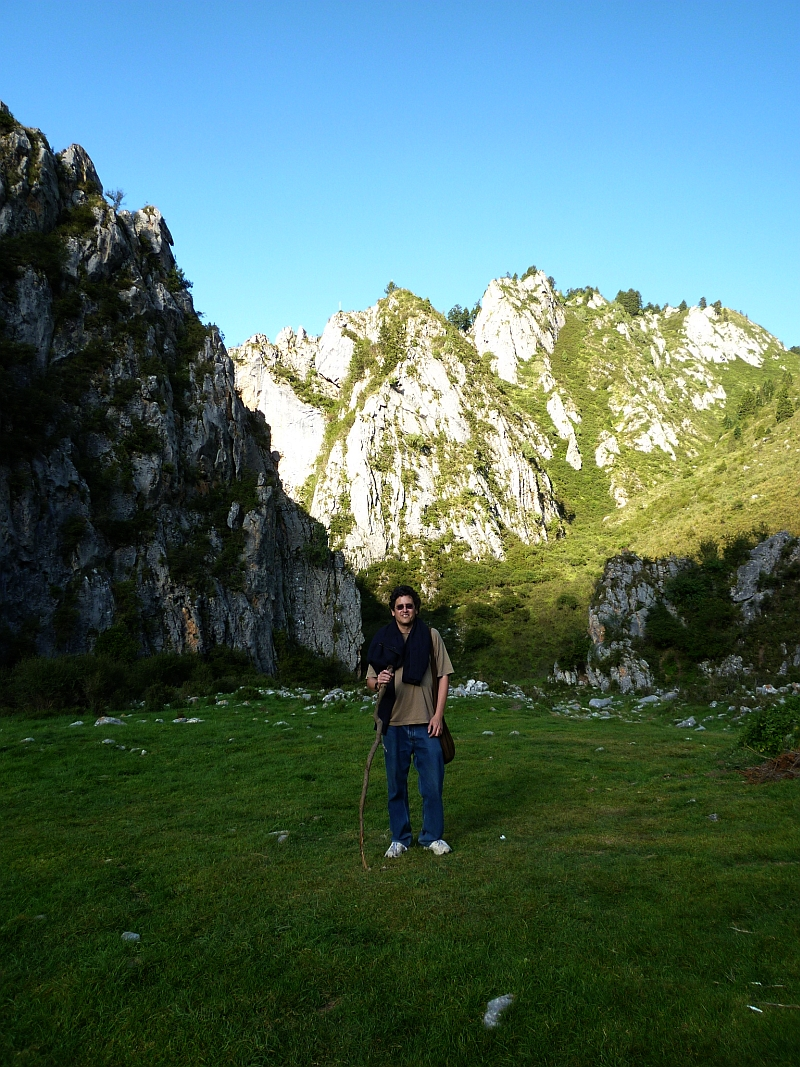But I also had lots of time sitting in trains, buses and automobiles to think about the $\mathrm{E}_8$ root polytope. Calculations along these lines turn out to be a great antidote to boredom!

So now I’d like to show you how to calculate the number of vertices, edges, 2d faces, etc. of this 8-dimensional polytope. What’s interesting is not the answers so much as the technique, which involves Dynkin diagrams.

If you take a bunch of equal-sized balls in 8 dimensions, and get as many as possible to all touch a central one, their centers will be the vertices of the $\mathrm{E}_8$ root polytope. Here are two attempts to draw it—click for more information:In its full 8-dimensional glory, this shape has:

• 240 vertices
• 6,720 edges
• 60,480 2d faces, which are all equilateral triangles
• 241,920 3d faces, which are all regular tetrahedra
• 483,840 4d faces, which are all regular 4-simplexes
• 483,840 5d faces, which are all regular 5-simplexes
• 207,360 6d faces, which are all regular 6-simplexes
• 19,440 7d faces, consisting of 17,280 regular 7-simplexes and 2,160 regular 7-orthoplexes

and

• a symmetry group with 696,729,600 elements, including rotations and reflections.

Remember, a 3-simplex is a tetrahedron: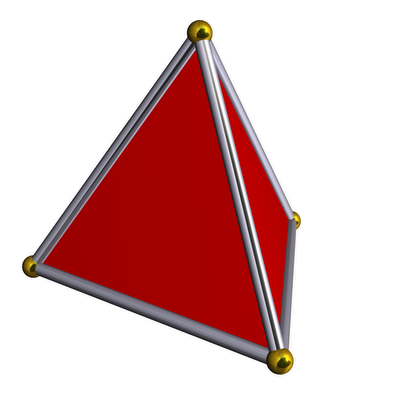while a 3-orthoplex is an octahedron: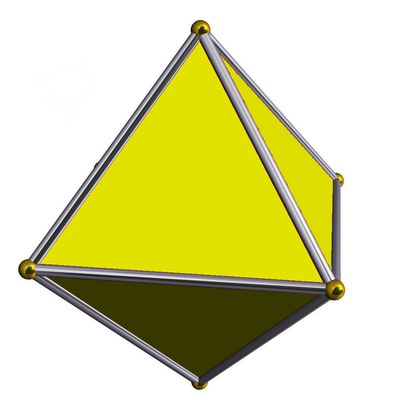Anyone can look up these crazy numbers on Wikipedia. But how can we see that they’re right?

That’s our challenge for today. It’s easiest if we define the $\mathrm{E}_8$ root polytope starting from this picture: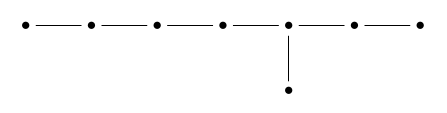and use the theory of simply-laced Dynkin diagrams, which are Dynkin diagrams without any multiple edges between dots. (Most of what I’m about to say generalizes to other Dynkin diagrams, but I’ll save a bit of time by focusing on this case.)

Suppose we’ve got such a diagram with $n$ dots. At an elementary level, this diagram tells us to take a bunch of unit vectors in $n$-dimensional Euclidean space, and make sure they lie at a $90^\circ$ angle if there’s no edge connecting them, and a $120^\circ$ angle if there is an edge connecting them.

We get a lattice called the root lattice by taking all integer linear combinations of these vectors. The nonzero vectors closest to the origin are called roots. These roots are the vertices of an $n$-dimensional convex polytope called the root polytope.

The roots include the vectors we started with, one for each dot in our Dynkin diagram: these are called the simple roots. But there are also a lot more.

Starting with the $\mathrm{E}_8$ Dynkin diagram, we can in principle figure out everything we want to know about the $\mathrm{E}_8$ root polytope, including how many faces of each dimension it has, and the size of its symmetry group. But to do such things elegantly, it helps to use some tricks.

### Coxeter groups

The main trick is to use the theory of Coxeter groups. Any simply-laced Dynkin diagram gives such a group, and it’s very easy to describe. It has one generator $s_i$ obeying

$s_i^2 = 1$

for each dot in the Dynkin diagram, one relation

$s_i s_j s_i = s_i s_j s_i$

for each pair of dots connected by an edge, and one relation

$s_i s_j = s_j s_i$

for each pair of dots not connected by an edge. Each generator $s_i$ corresponds to a reflection: the reflection that flips the $i$th simple root to its negative.

For example, starting with the Dynkin diagram called $\mathrm{A}_3$: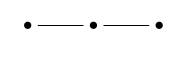we get a group with 3 generators, which happens to be the symmetry group of a regular tetrahedron:So, you should think of the three dots in the Dynkin diagram as standing for ‘vertex’, ‘edge’ and ‘face’. Why? Because the corresponding generators of the Coxeter group are reflections that:

• switch two neighboring vertices,

• switch two neighboring edges, and

• switch two neigboring faces,

respectively!

The $\mathrm{A}_n$ diagram gives the symmetry group of a regular $n$-simplex in just the same way. A similar story applies to the symmetry group of the regular $n$-dimensional orthoplex, but the Dynkin diagram for this is not simply laced, so I won’t get into the details. The cases we really need now are the $\mathrm{E}_8$ Dynkin diagram and the sub-diagrams of this.

For example, if we pull off the end dot of $\mathrm{E}_8$ we get a sub-diagram called $\mathrm{D}_7$, which looks like this: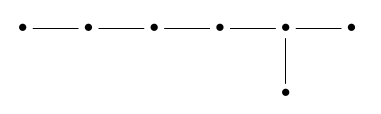or, after prettying it up, this: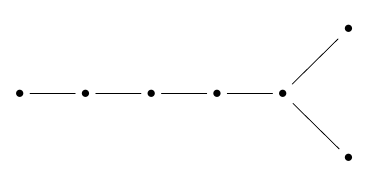Reading from left to right, can think of the dots here as standing for ‘vertex’, ‘edge’, ‘2d face’, ‘3d face’ and so on… but when we get to the right end of the diagram, obviously something funny must happen!

There are two dots that mean ‘top-dimensional face’. The reason is that this Coxeter group is the symmetry group of a 7-orthoplex that has its 6-dimensional faces colored alternately white and black. We only consider symmetries that carry faces to faces of the same color. Two 6d faces intersect in at most one 5d face, so there’s no dot for ‘5d face’. We can summarize the story like this: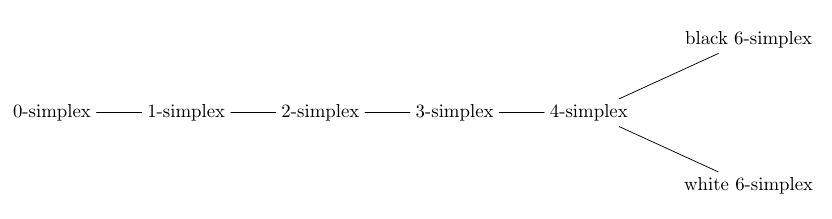All the $\mathrm{D}_n$ Dynkin diagrams look similar, with some number of dots in a row followed by two at the end… and they all work the same way. Their Coxeter groups are the symmetry groups of orthoplexes with their top-dimensional faces colored alternately white and black.

In the case of $\mathrm{E}_8$, something even stranger happens! The top-dimensional faces are of two kinds: 7-simplex and 7-orthoplex: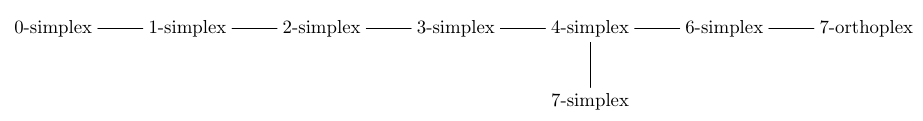Other subtleties arise for the sub-diagrams $\mathrm{E}_7$ and $\mathrm{E}_6$. So, you’re probably thinking that this subject is an elaborate quagmire. But it’s not.

### Coxeter complexes

To deal with all Coxeter groups in a systematic way, it’s better to think of them as symmetry groups of certain simplicial complexes called ‘Coxeter complexes’. Roughly speaking, a simplicial complex is a gadget made of 0-simplexes, 1-simplexes, 2-simplexes, 3-simplexes, and so on — all stuck together in a nice way.

If you have a Coxeter diagram with $n$ dots, the highest dimension of the simplexes in its Coxeter complex is $n-1$. There is one of these top-dimensional simplexes for each element of the Coxeter group. For example, I’ve already said this Dynkin diagram:gives the Coxeter group consisting of symmetries of a regular tetrahedron — by which I mean all reflections and rotations. This group has 4! = 24 elements, so the Coxeter complex is built from 24 triangles. And in fact, we get it by barycentrically subdividing the surface of a tetrahedron! We can draw it on a sphere, like this:You can see there are 24 triangles. And, you can see that if you fix a given triangle, there’s a unique symmetry of the Coxeter group mapping it to any other triangle.

In general, the Coxeter group of a Dynkin diagram with $n$ dots always acts as linear transformations of $\mathbb{R}^n$. Each root gives a reflection that flips that root to its negative. So, this group also acts on the $(n-1)$-sphere. If we take this sphere and chop it up along the hyperplanes corresponding to all the reflections in the Coxeter group, we get the Coxeter complex. See if you can visualize this in the picture above.

Even better, if you pick any top-dimensional simplex in the Coxeter complex, there always exactly one element of the Coxeter group that maps it to any other top-dimensional simplex. So the Coxeter complex is the best possible thing made out of simplexes on which the Coxeter group acts as symmetries!

The size of the Coxeter group corresponding to a Dynkin diagram is always the product of some integers, one for each dot in the Dynkin diagram. These integers have lots of important properties, and calculating them is really the key to all the problems we’ve set out to solve. To start with, let me just show you these numbers and what we can do with them.

Here they are. For any Dynkin diagram $D$, let $W(D)$ be its Coxeter group, also known as its Weyl group. Here are the sizes of these groups for all the simply-laced Dynkin diagrams:

$\begin{array}{ccll} |W(\mathrm{A}_n)| &=& 2 \cdot 3 \cdot 4 \cdot \cdots \cdot (n+1) &=& (n+1)! \\ |W(\mathrm{D}_n)| &=& 2 \cdot 4 \cdot 6 \cdot \cdots \cdot 2(n-1) \cdot n &=& (2n)?! \\ |W(\mathrm{E}_6)| &=& 2 \cdot 5 \cdot 6 \cdot 8 \cdot 9 \cdot 12 &=& 51,840 \\ |W(\mathrm{E}_7)| &=& 2 \cdot 6 \cdot 8 \cdot 10 \cdot 12 \cdot 14 \cdot 18 &=& 2,903,040 \\ |W(\mathrm{E}_8)| &=& 2 \cdot 8 \cdot 12 \cdot 14 \cdot 18 \cdot 20 \cdot 24 \cdot 30 &=& 696,729,600 \end{array}$

It’s easy to see that $W(A_n)$, the symmetry group of the $n$-dimensional simplex, has size equal to

$|W(\mathrm{A}_n)| = (n+1)!$

since the $n$-simplex has symmetries permuting its vertices any way you want. While it’s not a simply-laced example, it’s also easy to see that $W(B_n)$, the symmetry group of the $n$-dimensional orthoplex, has size equal to

$|W(\mathrm{B}_n)| = 2^n n!$

This number is equal to the double factorial

$(2n)!! = 2 \cdot 4 \cdot \cdots \cdot 2n$

Finally, it’s also easy to see that $W(D_n)$, the symmetry group of the orthoplex with its top-dimensional faces colored alternately white and black, is half as big. So, its size is the half double factorial of $2n$:

$(2n)?! = 2 \cdot 4 \cdots \cdot 2(n-1) \cdot n$

So, the only problem is computing the size of $W(\mathrm{E}_6)$, $W(\mathrm{E}_7)$, and $W(\mathrm{E}_8)$. I’ll talk about this later.

You might think it’s somewhat arbitrary how we wrote the size of these Coxeter groups as products of numbers—but it’s not! I’ll talk about this more at the end of the article. But here’s a little taste of the fun:

Puzzle 1. If you double each of these numbers and subtract one, then add up the results, you get the dimension of the Lie group corresponding to this Dynkin diagram. Why?

Let’s check that it works for $\mathrm{E}_8$:

$2 \cdot (2 + 8 + 12 + 14 +18 + 20 + 24 + 30) - 8 = 2 \cdot 128 - 8 = 248$

Yes, this is the dimension of the Lie group $\mathrm{E}_8$!

Assuming we know the size of the Coxeter groups for all the simply-laced Dynkin diagrams, let’s see if we can calculate how many faces of each kind the $\mathrm{E}_8$ root polytope has.

I explained the method in week187. Each Dynkin diagram $D$ describes a kind of ‘incidence geometry’ with different kinds of figures—points, edges, triangles, tetrahedra and so on—one for each dot in the Dynkin diagram. These figures can be ‘incident’ to each other—e.g., a triangle can lie on a tetrahedron—and there’s one basic incidence relation for each edge in the Dynkin diagram.

The Coxeter group $W(D)$ acts transitively on the figures of any given kind. We can also work out the subgroup of $W(D)$ that preserves a figure of a given kind. To do this, we just remove the corresponding dot from the Dynkin diagram, and get a sub-diagram $E \subset D$. Then $W(E) \subseteq W(D)$ is the subgroup we want!

So, the set of the figures of a given kind is $W(D)/W(E)$. Thus, the number of figures of this kind is the ratio

$\frac{|W(D)|}{|W(E)|}$

To see if you understand this, let’s do an easy example! How many edges does a tetrahedron have? Here our Dynkin diagram $D$ is $\mathrm{A}_3$:It describes a geometry, namely a tetrahedron, with figures of three kinds: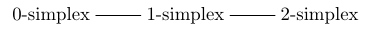Suppose we want to count the number of 1-simplexes, or edges. We take the ‘1-simplex’ dot: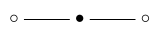and we remove it, obtaining this sub-diagram: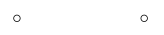Hmm, this isn’t connected! But that’s okay. This diagram is the disjoint union of two copies of the one-dot Dynkin diagram, $\mathrm{A}_1$. If you follow the rules, you’ll see its Coxeter group is the product

$W(\mathrm{A}_1) \times W(\mathrm{A}_1)$

So, the number of edges in a tetrahedron is

$\frac{|W(\mathrm{A}_3)|}{|W(\mathrm{A}_1)| \times |W(\mathrm{A}_1)|} = \frac{4!}{2! \times 2!} = 6$

which is right!

If you’ve never done this sort of stuff, I encourage you to play around with more examples. It’s lots of fun! But having spent years warming up with exercises like that, I’m now going to climb Mount Everest and count the various figures in the $\mathrm{E}_8$ root polytope.It starts out easy, but near the end, as you might imagine from this picture, it gets tricky—and I’ll slip and fall. Hanging from a icy cliff, I’ll ask for your help!

### 0-simplexes

If we remove the ‘vertex’ dot from the $\mathrm{E}_8$ Dynkin diagram: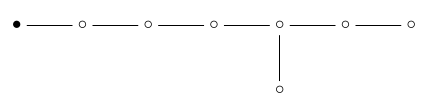we’re left with $\mathrm{E}_7$: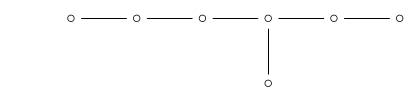So, the number of vertices of the $\mathrm{E}_8$ root polytope is

$\begin{array}{ccl} \frac{|W(\mathrm{E}_8)|}{|W(\mathrm{E}_7)|} &=& \frac{2 \cdot 8 \cdot 12 \cdot 14 \cdot 18 \cdot 20 \cdot 24 \cdot 30}{2 \cdot 6 \cdot 8 \cdot 10 \cdot 12 \cdot 14 \cdot 18} \\ &=& \frac{ 20 \cdot 24 \cdot 30}{6 \cdot 10} \\ &=& 240 \end{array}$

as we knew!

Just for fun, in the next to last step I cancelled all the numbers I could from the big fraction in the top line. This is worth doing because a $q$-deformed version of the same calculation lets us count the number of points in a certain space called a ‘Grassmannian’ for the version of the group $\mathrm{E}_8$ defined over the finite field with $q$ elements, where $q$ is any prime power. I explained how this works in week186 and week187.

In the $q$-deformed count we replace integers with $q$-integers, defined as follows:

$[n] = 1 + q + q^2 + \cdots + q^{n-1}$

So, for this particular Grassmannian the number of points is

$\frac{ \cdot  \cdot  \cdot  \cdot  \cdot  \cdot  \cdot }{ \cdot  \cdot  \cdot  \cdot  \cdot  \cdot }$

or, doing all the cancellations we can,

$\frac{  \cdot  \cdot }{ \cdot }$

This, believe it or not, is a polynomial in $q$. But we can’t say it’s equal to $$, because in general $[n m] \ne [n][m]$. Only when we set $q = 1$ does this equation hold, and then we get 240 points. So, our problem now, involving Coxeter groups, concerns the special case of the legendary but so far still mythical ‘field with one element’.

But if we wanted, we could look at the group $\mathrm{E}_8$ defined over the field with 2 elements. It acts in a transitive way on a set with

$\frac{  \cdot  \cdot }{ \cdot }$

points. How many points is that? In this case

$[n] = 1 + 2 + 4 + \cdots + 2^{n-1} = 2^n - 1$

so the number of points is

$\frac{(2^{20} - 1)(2^{24} - 1)(2^{30} - 1)}{(2^6 - 1)(2^{10} - 1)} = 293,091,386,578,365,375$

### 1-simplexes

If we remove the ‘edge’ dot from the $\mathrm{E}_8$ Dynkin diagram: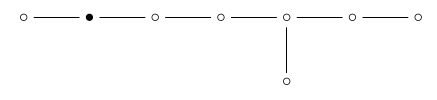we’re left with $\mathrm{A}_1 \times \mathrm{E}_6$: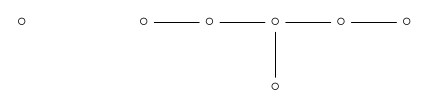So, the number of edges of the $\mathrm{E}_8$ root polytope is

$\begin{array}{ccl} \frac{|W(\mathrm{E}_8)|}{|W(\mathrm{A}_1)| \times |W(\mathrm{E}_6)|} &=& \frac{2 \cdot 8 \cdot 12 \cdot 14 \cdot 18 \cdot 20 \cdot 24 \cdot 30}{2 \times (2 \cdot 5 \cdot 6 \cdot 8 \cdot 9 \cdot 12)} \\ &=& \frac{14 \cdot 18 \cdot 20 \cdot 24 \cdot 30}{2 \cdot 5 \cdot 6 \cdot 9} \\ &=& 6,720 \end{array}$

### 2-simplexesA 2-simplex is just a triangle. If we remove the ‘2-simplex’ dot: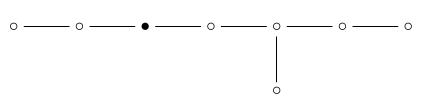we’re left with $\mathrm{A}_2 \times \mathrm{D}_5$: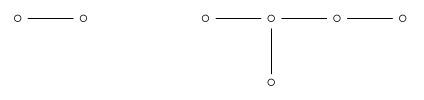so the number of 2d faces is

$\begin{array}{ccl} \frac{|W(\mathrm{E}_8)|}{|W(\mathrm{A}_2)| \times |W(\mathrm{D}_5)|} &=& \frac{2 \cdot 8 \cdot 12 \cdot 14 \cdot 18 \cdot 20 \cdot 24 \cdot 30}{(2 \cdot 3) \times (2 \cdot 4 \cdot 6 \cdot 8 \cdot 5)} \\ &=& \frac{12 \cdot 14 \cdot 18 \cdot 20 \cdot 24 \cdot 30}{2 \cdot 3 \cdot 4 \cdot 5 \cdot 6 \cdot 8}\\ &=& 60,480 \end{array}$

### 3-simplexesThis is a funny picture of a 3-simplex, or tetrahedron. If we remove the ‘3-simplex’ dot: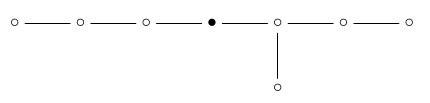we’re left with $\mathrm{A}_3 \times \mathrm{A}_4$: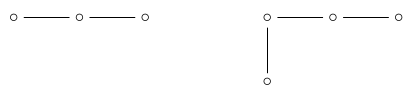so the number of 3d faces is

$\begin{array}{ccl} \frac{|W(\mathrm{E}_8)|}{|W(\mathrm{A}_3)| \times |W(\mathrm{A}_4)|} &=& \frac{2 \cdot 8 \cdot 12 \cdot 14 \cdot 18 \cdot 20 \cdot 24 \cdot 30}{(2 \cdot 3 \cdot 4) \times (2 \cdot 3 \cdot 4 \cdot 5)} \\ &=& \frac{8 \cdot 12 \cdot 14 \cdot 18 \cdot 20 \cdot 24 \cdot 30}{2 \cdot 3 \cdot 3 \cdot 4 \cdot 4 \cdot 5}\\ &=& 241,920 \end{array}$

### 4-simplexesThis is a picture of a 4-simplex. If we remove the ‘4-simplex’ dot from the $\mathrm{E}_8$ Dynkin diagram: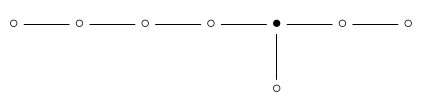we’re left with $\mathrm{A}_4 \times \mathrm{A}_1 \times \mathrm{A}_2$: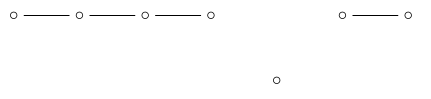so the number of 4d faces is

$\begin{array}{ccl} \frac{|W(\mathrm{E}_8)|}{|W(\mathrm{A}_4)| \times |W(\mathrm{A}_1)| \times |W(\mathrm{A}_4)|} &=& \frac{2 \cdot 8 \cdot 12 \cdot 14 \cdot 18 \cdot 20 \cdot 24 \cdot 30}{(2 \cdot 3 \cdot 4 \cdot 5) \times 2 \times (2 \cdot 3)} \\ &=& \frac{8 \cdot 12 \cdot 14 \cdot 18 \cdot 20 \cdot 24 \cdot 30}{2 \cdot 2 \cdot 3 \cdot 3 \cdot 4 \cdot 5} \\ &=& 483,840 \end{array}$

### 5-simplexesAt this point we meet a mystery: there is no ‘5-simplex’ dot in the $\mathrm{E}_8$ Dynkin diagram!This is a bit like how there is no 5-simplex dot in the $\mathrm{D}_7$ Dynkin diagram:The resolution there is that if we have ‘complete flag’ consisting of one figure of each kind shown in this picture, all incident, there is a unique 5-simplex that’s incident to all the other figures. Indeed, there’s at most one 5-simplex touching any pair consisting of a white and a black 6-simplex. So, the 5-simplex is ‘redundant’.

The same sort of resolution must, I think, apply to $\mathrm{E}_8$. Understanding this in detail would make a good test of our understanding. But let’s move on!

### 6-simplexesIf we remove the ‘6-simplex’ dot: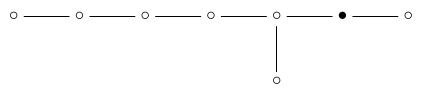we’re left with $\mathrm{A}_6 \times \mathrm{A}_1$: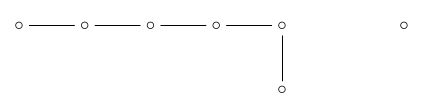so the number of 6d faces is apparently:

$\begin{array}{ccl} \frac{|W(\mathrm{E}_8)|}{|W(\mathrm{A}_6)| \times |W(\mathrm{A}_1)| } &=& \frac{2 \cdot 8 \cdot 12 \cdot 14 \cdot 18 \cdot 20 \cdot 24 \cdot 30}{(2 \cdot 3 \cdot 4 \cdot 5 \cdot 6 \cdot 7) \times 2} \\ &=& \frac{8 \cdot 12 \cdot 14 \cdot 18 \cdot 20 \cdot 24 \cdot 30}{2 \cdot 3 \cdot 4 \cdot 5 \cdot 6 \cdot 7} \\ &=& 69,120 \end{array}$

But this does not agree with Wikipedia’s answer! It says our polytope has 207,360 6-dimensional faces, which are all regular 6-simplexes. So, we have another mystery on our hands here, which we will return to.

### 7-simplexesIf we remove the ‘7-simplex’ dot: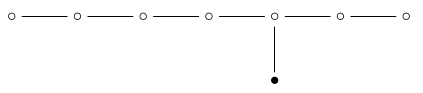we are left with $\mathrm{A}_7$: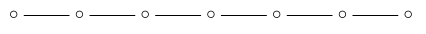so the number of 7-dimensional simplex faces is:

$\begin{array}{ccl} \frac{|W(\mathrm{E}_8)|}{|W(\mathrm{A}_7)| } &=& \frac{2 \cdot 8 \cdot 12 \cdot 14 \cdot 18 \cdot 20 \cdot 24 \cdot 30}{2 \cdot 3 \cdot 4 \cdot 5 \cdot 6 \cdot 7 \cdot 8} \\ &=& \frac{12 \cdot 14 \cdot 18 \cdot 20 \cdot 24 \cdot 30}{3 \cdot 4 \cdot 5 \cdot 6 \cdot 7} \\ &=& 17,280 \end{array}$

### 7-orthoplexesIf we remove the ‘7-orthoplex’ dot: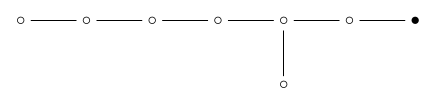we are left with $\mathrm{D}_7$: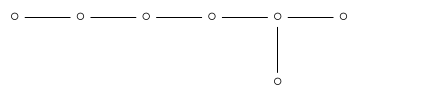so the number of 7-dimensional orthoplex faces is:

$\begin{array}{ccl} \frac{|W(\mathrm{E}_8)|}{|W(\mathrm{D}_7)| } &=& \frac{2 \cdot 8 \cdot 12 \cdot 14 \cdot 18 \cdot 20 \cdot 24 \cdot 30}{2 \cdot 4 \cdot 6 \cdot 8 \cdot 10 \cdot 12 \cdot 7} \\ &=& \frac{12 \cdot 14 \cdot 18 \cdot 20 \cdot 24 \cdot 30}{4 \cdot 6 \cdot 7 \cdot 10 \cdot 12} \\ &=& 2,160 \end{array}$

### Further mysteries

We’ve got some mysteries to solve.

#### 6-simplexes

First, our calculations seemed to show the number of 6-simplex faces of root polytope of $\mathrm{E}_8$ is 69,120, while Wikipedia says the answer is 207,360. Luckily we can compute the answer another way, which sheds a little light on this problem.

Our polytope has 17,280 7-simplex faces and 2,160 7-orthoplex faces. Each 7-simplex has 8 faces, all of which are 6-simplexes. Each 7-orthoplex has 27 faces, all of which are 6-simplexes. So, we could naively count the total number of 6-simplexes and get

$17,280 \cdot 8 + 2,160 \cdot 2^7 = 138,240 + 276,480 = 414,720$

But this is double-counting, since each 6-simplex touches two 7-simplexes. (The top-dimensional faces of a convex polytope always meet in pairs along faces of the next highest dimension.) So the real count is half this:

$\frac{138,240}{2} + \frac{276,480}{2} = 69,120 + 138,240 = 207,360$

This matches the answer on Wikipedia. But it also sheds some light on our wrong answer. Our wrong answer, 69,120, is exactly the total number of faces of all the 7-simplexes. It’s also half the total number of faces of the 7-orthoplexes. It’s also exactly 1/3 of the correct answer!

All this suggests that the 6-simplex faces of our polytope come in two kinds, which cannot be interchanged by symmetries of the polytope. 1/3 of them are the ones we counted, the kind mentioned in this diagram:2/3 of them are 6-simplexes of some other kind, which do not appear in the above diagram.

If this seems weird, remember that this diagram also does not include the 5-simplex faces of our polytope! Also remember that the $\mathrm{D}_7$ diagram, corresponding to the 7-orthoplex, gives another example where some simplexes come in two kinds, while others do not appear at all, even though they exist as faces of the 7-orthoplex:Even if this is true, it’s clearly not enough for a full understanding. If our polytope has two kinds of 6-simplex as faces, how do they differ? And where is the number 1/3 coming from?

Here’s my guess. The 7-orthoplex faces of our polytope have 6-simplex faces that can be colored alternately white and black, and all the symmetries of our polytope preserve this two-coloring. As evidence, note that the symmetries preserving a 7-orthoplex form the group $\mathrm{D}_7$, which preserves such a two-coloring. But I’m guessing we can do this two-coloring in a consistent way, so every 6-simplex in our polytope is either white or black.

And I’m guessing we can do this so that each 7-orthoplex touches another 7-orthoplex on each of its black faces, and a 7-simplex on each of its white faces! On the other hand, every 7-simplex is completely surrounded by 7-orthoplexes.

If we can do this:

• Each white 6-simplex is the face of a unique 7-simplex, so the number of white 6-simplexes is

$17,280 \cdot 8 = 138,240$

These are the 6-simplexes we counted.

• Each white 6-simplex is the face of a unique 7-orthoplex, so the number of white 6-simplexes is also

$\frac{2,160 \cdot 2^7}{2} = 138,240$

where we get a factor of $1/2$ because only half the 6-simplex faces of each 7-orthoplex are white. This is a consistency check!

• Each black 6-simplex is the face of two 7-orthoplexes, so the number of black 6-simplexes is

$\frac{2,160 \cdot 2^7}{4} = 69,120$

• The total number of 6-simplexes is

$69,120 + 138,240 = 207,360$

as it says on Wikipedia.

All this fits together nicely… so while I haven’t proved the existence of this ‘consistent two-coloring’ and this arrangement of 6-simplexes and 6-orthoplexes, I believe it.

Puzzle 2. Prove or disprove my guesses here!

#### 5-simplexes

Why does our polytope have 483,840 5d faces, which are all regular 5-simplexes? I haven’t figured out how to count them. With work I should be able to do it using my guesses in the previous section, but I made these guess just a few minutes ago.

Notably, 483,840 is also the number of 4d faces.

Puzzle 3. Show how to count the 5d faces of the $\mathrm{E}_8$ root polytope.

#### The magic numbers

All my calculations rely on general results that I understand, together with some numbers:

$\begin{array}{ccll} W(\mathrm{E}_6)| &=& 2 \cdot 5 \cdot 6 \cdot 8 \cdot 9 \cdot 12 &=& 51,840 \\ |W(\mathrm{E}_7)| &=& 2 \cdot 6 \cdot 8 \cdot 10 \cdot 12 \cdot 14 \cdot 18 &=& 2,903,040 \\ |W(\mathrm{E}_8)| &=& 2 \cdot 8 \cdot 12 \cdot 14 \cdot 18 \cdot 20 \cdot 24 \cdot 30 &=& 696,729,600 \end{array}$

In fact for my calculations I only need to know the sizes of these three Coxeter groups, but it’s irresistible to try understanding the ‘magic numbers’ that you can multiply to get their sizes.

Puzzle 4. What’s the best way to calculate these magic numbers?

Back in week186 and week187 I gave many characterizations of these magic numbers. Unfortunately, they don’t seem to make it easy to calculate these numbers for $\mathrm{E}_6, \mathrm{E}_7$ and $\mathrm{E}_8$.

I bet somebody knows an easy way. But to help you with this puzzle, I’ll tell you eight hard ways!

Start with any Dynkin diagram $D$, not necessarily simply-laced. Let $G(\mathbb{F})$ be the corresponding simple algebraic group over a field $\mathbb{F}$. In particular,

$G = G(\mathbb{C})$

is a complex Lie group. Suppose the diagram $D$ has $n$ dots. Then the magic numbers $d_1, \dots, d_n$ are natural numbers such that

$|W(D)| = d_1 \cdot \cdots \cdot d_n$

Moreover:

1) The real cohomology of $G$ is an exterior algebra on generators $x_1, \dots, x_n$, one for each dot of the Dynkin diagram. The degrees of these generators are all odd, and the degree of $x_i$ is $2d_i - 1$.

2) The real cohomology of the classifying space $B G$ is a polynomial algebra on generators $y_1, \dots, y_n$. The degrees of these generators are all even, and the degree of $y_i$ is $2 d_i$.

3) The Weyl group $W(D)$ acts on $\mathbb{R}^n$. The algebra of polynomials on $\mathbb{R}^n$ invariant under this group action is a free commutative algebra on generators $z_1, \dots, z_n$. The polynomial $z_i$ is homogeneous of degree $d_i$.

4) Define the q-polynomial of the Dynkin diagram $D$ to be the polynomial in $q$ where the coefficient of $q^k$ is the number of $k$-cells in the Bruhat decomposition of the flag variety $G/B$. Here $B$ is the Borel subgroup of $G$, and the Bruhat decomposition is a standard way of writing $G/B$ as disjoint union of $k$-cells, that is, copies of $\mathbb{C}^k$. Then the $q$-polynomial equals

$[d_1] \cdot \cdots \cdot [d_n]$

where $[d_i] = 1 + q + \cdots + q^{d_i}$.

5) If the coefficient of $q^k$ in the $q$-polynomial is $d$, the $(2i)$th homology group of $G/B$ is $\mathbb{Z}^d.$

6) The value of the $q$-polynomial when $q$ is a prime power is the cardinality of $G(\mathbb{F}_q)/B(\mathbb{F}_q)$. Here $\mathbb{F}_q$ is the field with $q$ elements, and $B(\mathbb{F}_q)$ is the Borel subgroup of the simple algebraic group $G(\mathbb{F}_q)$.

7) The coefficient of $q^k$ in the $q$-polynomial is the number of Coxeter group elements of length $k$. Here the length of any element in the Coxeter group is its minimal length as a word when we write it as product of the generators $s_1, \dots, s_n$ corresponding to dots in the Dynkin diagram.

8) The coefficient of $q^k$ in the polynomial is the number of top-dimensional simplexes of distance $k$ from a chosen top-dimensional simplex in the Coxeter complex. Here we measure ‘distance’ between top-dimensional simplexes in the hopefully obvious way, based on how many walls you need to cross to get from one to the other.

If you know enough stuff you may have fun proving all these descriptions of the magic numbers are equivalent.

I have not managed to use any of these descriptions of the magic numbers to solve this problem:

Puzzle 5. Why is these sequence of magic numbers for any Dynkin diagram ‘symmetrical’?

To explain what I mean here, look at the pattern of spacings between magic numbers for $\mathrm{E}_6$:

$\begin{array}{cccccccccccc} \mathbf{2} & & & \mathbf{5} & \mathbf{6} & & \mathbf{8} & \mathbf{9} & & & \mathbf{12} \\ \bullet & \circ & \circ & \bullet & \bullet & \circ & \bullet & \bullet & \circ & \circ &\bullet \end{array}$

It doesn’t change when you reflect it! The same is true for $\mathrm{E}_7, \mathrm{E}_8$ and also the non-exceptional cases if you write the magic numbers in increasing order. Why? It’s reminiscent of Poincaré duality, but I don’t see how it is that.

Puzzles 1 and 5 could be clues for Puzzle 4.

Posted at September 3, 2013 4:19 AM UTC

TrackBack URL for this Entry:   https://golem.ph.utexas.edu/cgi-bin/MT-3.0/dxy-tb.fcgi/2653

### Re: Integral Octonions (Part 5)

I’ll need to think about it more, but I’m pretty sure that (1) the symmetry is more evident for the magic numbers minus 1, and (2) they’re the exponents appearing in the eigenvalues of the Coxeter element. Then the symmetry comes from that guy being an orthogonal transformation of Lie(T).

There’s a lot more numerology about these exponents/degrees, and the place to read it is gray Humphreys (“Reflection groups and Coxeter groups”), which I don’t have with me.

Posted by: Allen K. on September 3, 2013 5:02 AM | Permalink | Reply to this

### Re: Integral Octonions (Part 5)

Thanks! I’ll have to look at gray Humphreys again.

Here’s a nice thing about the magic numbers minus 1: their sum is the number of positive roots of our Lie algebra! So, I was hoping someone like you could tell me how to partition the positive roots in some nice way that would let me calculate the magic numbers.

If this were possible, the ‘symmetry’ of the magic numbers could be a clue as to how.

But how can you take the positive roots of:

$\mathrm{A}_2$ and partition them into bunches of size 1 and 2,

$\mathrm{B}_2$ and partition them into bunches of size 1 and 3,

$\mathrm{G}_2$ and partition them into bunches of size 1 and 5,

$\mathrm{A}_3$ and partition them into bunches of size 1, 2 and 3,

$\mathrm{B}_3$ and partition them into bunches of size 1, 3 and 5,

etc.?

Posted by: John Baez on September 3, 2013 9:37 AM | Permalink | Reply to this

### Re: Integral Octonions (Part 5)

“Height” of the root, = sum of the coefficients when expanded in simple roots. Or, you could call it the weight multiplicities for the action of a principal SL(2).

Which shows you there’s a definition of these numbers for any representation, not just the adjoint representation!

Incidentally, under the geometric Satake correspondence telling you that each irrep Vλ = IH(Grλ inside the affine Grassmannian for the Langlands dual group), the decomposition into weight spaces for the principal SL(2) is exactly the grading on intersection homology.

Posted by: Allen K. on September 3, 2013 3:07 PM | Permalink | Reply to this

### Re: Integral Octonions (Part 5)

John wrote:

Here’s a nice thing about the magic numbers minus 1: their sum is the number of positive roots of our Lie algebra! So, I was hoping someone like you could tell me how to partition the positive roots in some nice way that would let me calculate the magic numbers.

If this were possible, the ‘symmetry’ of the magic numbers could be a clue as to how.

But how can you take the positive roots of:

$A_2$ and partition them into bunches of size 1 and 2,

$B_2$ and partition them into bunches of size 1 and 3,

[etc.]

Allen wrote:

“Height” of the root, = sum of the coefficients when expanded in simple roots.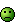I’d considered that, but that doesn’t do the job, does it? For a rank $n$ group, I need a partition of its positive roots into $n$ parts, the size of each being one less than ‘magic number’.

For $A_2$ we have 2 positive roots of height one and 1 of height two… so we get the partition (2,1), which looks good, since the magic numbers are (3,2).

But for $B_2$ we have 2 of height one, 1 of height two and 1 of height three, so we get the partition (2,1,1). But we want the partition (3,1), since the magic numbers in this case are (4,2).

Am I confused?

Posted by: John Baez on September 4, 2013 3:25 AM | Permalink | Reply to this

### Re: Integral Octonions (Part 5)

I was not confused. Walking home I made an outrageous conjecture. I was hoping to become famous for having such a crazy but true idea. However, it turns out to be a theorem in Section 3.20 of Humphrey’s gray book.

To calculate the magic numbers, you calculate how many positive roots of each height there are, and use this to get a partition of the total number of positive roots, just like Allen said. For example, $\mathrm{B}_2$ has 2 positive roots of height one, 1 of height two and 1 of height three, so we get the partition (2,1,1).

But then you write this partition as a Young diagram:

XX
X
X


Then you reflect this Young diagram and get another Young diagram:

XXX
X



Then you read this as a partition, namely (3,1). And finally, you add one to each of these numbers, and you get the magic numbers! For $\mathrm{B}_2$ you get (4,2). But the procedure works in general for any Dynkin diagram.

This is insane, but it’s true.

Posted by: John Baez on September 4, 2013 1:36 PM | Permalink | Reply to this

### Re: Integral Octonions (Part 5)

Allen is correct; this symmetry is Lemma 3.16 in Humphreys. The quick explanation is that $\zeta^{d_i-1}$ for $\zeta$ a particular $h$-th root of unity is the set of eigenvalues of the Coxeter element. Since this is a real matrix, its eigenvalues come in complex conjugate pairs. Here $h$ is the Coxeter number, the order of the Coxeter element.

Posted by: Ben Webster on September 3, 2013 3:43 PM | Permalink | Reply to this

### Re: Integral Octonions (Part 5)

Okay, Humphreys’ gray book Reflection Groups and Coxeter Groups is indeed an repository of occult wisdom on what I’m calling the ‘magic numbers’.

For example, in Section 3.20 he proves this:

Proposition. If $h$ is the Coxeter number of our Dynkin diagram $D$ and $1 \le m \le h$ is relatively prime to $h$, then $m+1$ is a magic number for $D$.

Recall that the Coxeter number is the number of roots divided by the number of dots in the Dynkin diagram. (For other descriptions, click the link!)

The dimension of the Lie algebra $\mathrm{E}_8$ is 248, and its Dynkin diagram has 8 dots, so the number of roots is 248 - 8 = 240, and its Coxeter number is 240/8 = 30. The numbers between 1 and 30 that are relatively prime to 30 are:

$1, 7, 11, 13, 17, 19, 23, 29$

So, adding one, we get some magic numbers for $\mathrm{E}_8$:

$2, 8, 12, 14, 18, 20, 24, 30$

But there are eight of them, and there’s always just one magic number for each dot in the Dynkin diagram, so these must be all the magic numbers!

This is black magic, and even the normally imperturbable Humphreys gives it an exclamation mark.

By the way, did you notice something funny about these numbers:

$1, 7, 11, 13, 17, 19, 23, 29 ?$

Except for 1, they’re all prime! 30 just happens to be the largest number for which every number besides 1 that’s smaller than it and relatively prime to it is actually prime. I don’t know if this is important here, but it’s darn suspicious.

For $\mathrm{E}_7$ we have to work harder. The dimension of the Lie algebra $\mathrm{E}_7$ is 133, so the number of roots is 133 - 7 = 126, and the Coxeter number is 126 / 7 = 18. Here are the numbers smaller than 18 that are relatively prime to 18:

$1, 5, 7, 11, 13, 17$

Adding one, we get magic numbers for $\mathrm{E}_7$:

$2, 6, 8, 12, 14, 18$

Unfortunately we only get six of the seven magic numbers this way. Luckily, Humphreys also proved that the sequence of magic numbers must be ‘symmetrical’ in the way I described. The only possibility is that the seventh magic number is 10:

$\begin{array}{ccccccccccccccccc} 2 &&&& 6 &&8 &&10 && 12 &&14 &&&& 18 \\ \bullet &\circ &\circ &\circ & \bullet &\circ &\bullet &\circ &\bullet &\circ &\bullet &\circ &\bullet &\circ&\circ &\circ& \bullet \end{array}$

By the way, did you notice anything funny about these numbers:

$1, 5, 7, 11, 13, 17 ?$

Yup!

For $\mathrm{E}_6$ we must work even harder for our supper, but I’ll leave that as a puzzle for you! To get you started, I’ll remind you that the dimension of the $\mathrm{E}_6$ is 78, so the number of roots is 78 - 6 = 72, so the Coxeter number is 72/6 = 12.

Puzzle 1 may come in handy here.

Posted by: John Baez on September 4, 2013 1:11 PM | Permalink | Reply to this

### Re: Integral Octonions (Part 5)

So, we’ve seen that every integer $1 < m < 30$ that’s relatively prime to 30 is actually prime. We’ve seen that these numbers are deeply related to the Weyl group $\mathrm{E}_8$.

We’ve seen that every integer $1 < m < 18$ that’s relatively prime to 18 is actually prime, and that these numbers are deeply related to the Weyl group of $\mathrm{E}_7$.

And if you did my last little puzzle, you’ll have seen that every integer $1 < m < 12$ that’s relatively prime to 12 is actually prime, and that these numbers are deeply related to the Weyl group of $\mathrm{E}_6$.

Numbers with this property are called very round, and the only very round numbers are

$1, 2, 3, 4, 6, 8, 12, 18, 24, 30$

Is the very roundness of 24 related to other interesting things about this famous number? Wikipedia points out:

The divisors of 24 — namely, $\{1, 2, 3, 4, 6, 8, 12, 24\}$ — are exactly those $n$ for which every invertible element of the commutative ring $\mathbb{Z}/n\mathbb{Z}$ is a square root of 1. Thus the multiplicative group $\mathbb{Z}/24\mathbb{Z} = \{\pm 1, \pm 5, \pm 7, \pm 11\}$ is isomorphic to the additive group $(\mathbb{Z}/2\mathbb{Z})^3$. This fact plays a role in Monstrous Moonshine.

Unfortunately it does not explain this remark! The best explanation I’ve found lies in Noam Elkies’ remarks in the Addendum to “week172”.

Now I’m wondering if there is an important Lie group which is related to 24 in the same way that $\mathrm{E}_8$, $\mathrm{E}_7$ and $\mathrm{E}_6$ are related to 30, 18 and 12.

Naively we’d be looking for a simple Lie group whose Coxeter number is 24 and whose magic numbers include the numbers you get by taking the numbers less than 24 and relatively prime to it, and adding 1:

$1+1, 5+1, 7+1 , 11+1, 13+1, 17+1, 19+1, 23+1$

This list of numbers is symmetrical about its mean, which is a property any list of magic numbers must have. If I’m not making a mistake, the only simple Lie groups with Coxeter number 24 are $\mathrm{A}_{11} = \mathrm{SL}(23)$, $\mathrm{B}_{12} = \mathrm{SO}(25)$, $\mathrm{C}_{12} = \mathrm{Sp}(24)$, and $\mathrm{D}_{13} = \mathrm{SO}(26)$. I think all of these have magic numbers including the above list. Of these, I guess $\mathrm{SO}(26)$ looks the most interesting.

Posted by: John Baez on September 5, 2013 6:36 AM | Permalink | Reply to this

### Re: Integral Octonions (Part 5)

Over on G+, Layra Idrani wrote:

You can prove the black-white thing by example, or at least I did.

Letting our vertices be

(±1,±1,0,0,0,0,0,0)

and permutations, and

(±1/2,±1/2,±1/2,±1/2,±1/2,±1/2,±1/2,±1/2)

with an even number of -1/2s, we get that a pair of vertices are connected by an edge iff their inner product is 1.

We can look at the following orthoplex:

(1,1,0,0,0,0,0,0)

(1,0,1,0,0,0,0,0)

(1,0,0,1,0,0,0,0)

(1,0,0,0,1,0,0,0)

(1,0,0,0,0,1,0,0)

(1,0,0,0,0,0,1,0)

(1,0,0,0,0,0,0,1)

(1,-1,0,0,0,0,0,0)

(1,0,-1,0,0,0,0,0)

(1,0,0,-1,0,0,0,0)

(1,0,0,0,-1,0,0,0)

(1,0,0,0,0,-1,0,0)

(1,0,0,0,0,0,-1,0)

(1,0,0,0,0,0,0,-1)

Now we figure out which sets of seven can be extended to a simplex via adding another vertex.

Consider a vertex (1,-1,0,0,0,0,0,0). For another vertex to have an edge to it, i.e. to have inner product 1, its first entry must be positive or 0, and its second entry must be negative or 0, since otherwise there would be a negative term in the inner product; both entries can’t be 0, and if both are non-zero then they must be 1/2 and -1/2 respectively.

Similarly, for any other vertex $v$ in the orthoplex, any vertex $w$ connected to $v$ must either match the sign of $v$ in precisely one of the two non-zero entries of $v$, or $w$ must match both and all of its coordinates must have magnitude 1/2.

We take a set of seven vertices in our orthoplex such that except for the first entry, if $v$ has a non-zero entry somewhere then the other 6 vertices have 0 there. These are all our 6-dimensional simplices in our orthoplex, since any other set would contain a pair of vertices whose inner product is 0. Suppose that of these, $\{v_i\}$ for $i$ from 1 to $k$ each have a -1 in position $p_i$. The point that has coordinate -1/2 in position $p_i$ and 1/2 in all other positions is of distance 2 from all the vertices given. Such a point is a vertex iff $k$ is even. So that gives us 64 6-simplices that extend to 7-simplices. Moreover, these 6-simplices alternate with the remaining 6-simplices, since given a 6-simplex with an even number of vertices that have a -1 in them, changing any of the vertices such that we still get a 6-simplex in the orthoplex yields a 6-simplex with an odd number of vertices with a -1 in them, and vice versa.

Now we just need to show that the other 64 6-simplices don’t extend to 7-simplices. Consider a set of seven vertices in the orthoplex such that an odd number have an entry of -1 and the seven vertices form a 6-simplex.

Suppose we have a vertex $w$ with a 0 entry. If it has 1 as its first entry, then it must have an entry of 1 or -1 somewhere else, and thus either matches or is orthogonal to a vertex in our 6-simplex. If $w$ has 0 as its first entry, then it must have non-zero entries in two other places, but as there are then five remaining entries, there is a vertex in our 6-simplex that is 0 in those two places and hence is orthogonal to $w$. If $w$ has only entries of ±1/2, then it must match each vertex in our simplex wherever that simplex is non-zero, but doing so yields a point with an odd number of entries of -1/2, which is not a vertex. So these 6-simplices cannot be extended to 7-simplices, and hence must be connected to orthoplexes (orthoplices?).

So this particular orthoplex has precisely half of its 6-faces connected to simplices, and the other half to other orthoplexes, and such faces alternate. By symmetry, this applies to all the orthoplexes.

Posted by: John Baez on September 3, 2013 12:11 PM | Permalink | Reply to this

### Re: Integral Octonions (Part 5)

Layra wrote:

These are all our 6-dimensional simplices in our orthoplex, since any other set would contain a pair of vertices whose inner product is 0. Suppose that of these, $\{ v_i\}$ for $i$ from 1 to $k$ each have a -1 in position $p_i$.

I don’t understand this. Is $v_i$ the $i$th component of a vertex, or the $i$th vertex? And what does ‘position $p_i$’ mean? What’s $p$? Or maybe by ‘a -1 in position $p_i$’ you mean that the $i$th component of the vector $v$ is -1? Or maybe the $i$th component of the vector $v_i$ is $-1$? I’m lost….

Posted by: John Baez on September 5, 2013 3:30 PM | Permalink | Reply to this

### Re: Integral Octonions (Part 5)

The pi are just numbers from 2 to 8, and the intended construction is for the pi-th component of the vector v_i to be -1.

Posted by: Layra on September 10, 2013 11:23 PM | Permalink | Reply to this

### Re: Integral Octonions (Part 5)

On other aspects of your octonion interests, such as here, did you see the recent Super Yang-Mills, division algebras and triality?

Posted by: David Corfield on September 4, 2013 9:52 AM | Permalink | Reply to this

### Re: Integral Octonions (Part 5)

I’d heard rumors of it but hadn’t seen it—thanks. In SUSY1 John Huerta and I described how to get a super-Yang–Mills theory from one division algebra: an idea not new to us, but we had to explain it to understand it. That idea must be a special case of this one, where they get a super-Yang–Mills theory from two division algebras.

The idea of combining two division algebras is fundamental to the magic square description of exceptional Lie algebras. It would be fun if that were related to this new work: it would suggest that the biggest, best Yang–Mills theory, the $\mathbb{O}, \mathbb{O}$ theory in ten dimensions, is related to $\mathrm{E}_8$—since that’s the case that gives $\mathrm{E}_8$ in the magic square.

I wish I could get to the bottom of this stuff, but it seems like a bottomless pool that’s somehow both dazzlingly beautiful and murky. What does it all mean? It’s as if our civilization is lacking some concepts that would let us answer that question.

Posted by: John Baez on September 4, 2013 10:47 AM | Permalink | Reply to this

### Re: Integral Octonions (Part 5)

So maybe their ten super Yang-Mills theories correspond to the upper (or lower) triangle of the magic square. Wasn’t part of the ‘magic’ of the magic square to do with the symmetry of the entries?

Posted by: David Corfield on September 4, 2013 11:51 AM | Permalink | Reply to this

### Re: Integral Octonions (Part 5)

Ah, no. They talk about their existing work – A magic square from Yang-Mills squared – on the magic square, and want to create a ‘magic pyramid’ on it as a base.

Posted by: David Corfield on September 4, 2013 11:55 AM | Permalink | Reply to this

### Re: Integral Octonions (Part 5)

Posted by: David Corfield on March 17, 2014 10:33 PM | Permalink | Reply to this

### Re: Integral Octonions (Part 5)

Your theory as to what the naive counting method was actually counting for the 6-simplices was bothering me, so I checked the calculation and you actually lost a division by 2.

2⋅8⋅12⋅14⋅18⋅20⋅24⋅30/(2!⋅7!) = 69,120

which makes a lot more sense since the 6-simplex node indicates 6-simplices that extend to a 7-orthoplex but not to a 7-simplex, otherwise there ought to be a line connecting the 6-simplex node to the 7-simplex node, which is what tipped me off.

Not having been previously aware of this method before, I actually panicked for a while thinking that I had grievously misunderstood.

In terms of the 5-simplices, the fastest way I can think to do it is to count the number of 5-simplices per 7-simplex (28) and then multiply by the number of 7-simplices (17280). This gives the right answer. Now we just have to show that this is the correct calculation to do.

We know that we only have to worry about 5-simplices that are contained in 7-simplices, since for any 5-simplex in an orthoplex, it can extend to a white 6-simplex, and uniquely so.

To show that each 5-simplex is in a unique 7-simplex, we look at 4-simplices. According to the diagram, each 4-simplex A is contained in exactly two 7-simplices, denoted B and C. Hence if a 5-simplex D contains A, then it can only be contained in B or C, no other 7-simplices, and indeed must be contained in at least one of B or C. Since all 5-simplices are identical, if any 5-simplex is in two 7-simplices, each 5-simplex must be in two 7-simplices.

So we assume that D is in both B and C. Since any other D’ that contains A must be in B or C, and D is in two simplices, D’ must also be in both B and C. We know that D’ exists since B has more than one vertex that isn’t in A.

D and D’ share 5 vertices and are both in the same 7-simplex B, and so thus together define a unique 6-simplex, and that 6-simplex is contained in B. But by assumption, they’re also both in C, and so that same 6-simplex is also in C. Thus we get that B and C share a 6-simplex, which is impossible.

Hence D is in exactly one of B and C, and similarly every 5-simplex is in precisely one 7-simplex.

Posted by: Layra Idarani on September 4, 2013 10:36 PM | Permalink | Reply to this

### Re: Integral Octonions (Part 5)

Layra wrote:

Your theory as to what the naive counting method was actually counting for the 6-simplices was bothering me, so I checked the calculation and you actually lost a division by 2:

2⋅8⋅12⋅14⋅18⋅20⋅24⋅30/(2!⋅7!) = 69,120

Oh! Good catch! One great thing about blogging is that people catch your mistakes… but in this case, it took real concentration to notice that mistake. Thanks!

Also thanks for helping me count the mysterious 5-simplexes.

According to the diagram, each 4-simplex A is contained in exactly two 7-simplices, denoted B and C.

How do you get that from the diagram? I assume you mean this diagram:Posted by: John Baez on September 5, 2013 6:56 AM | Permalink | Reply to this

### Re: Integral Octonions (Part 5)

Okay, so technically I don’t actually know that the diagram says what I think it does. My general heuristic for how the naive counting method works is that the entire diagram corresponds to complete flags, and that the subdiagrams correspond to partial flags. So that when you look at a dot, it’s really saying “take a shape that corresponds to this dot; removing the dot gives you a diagram of which one part corresponds to flags contained in that shape, and the remaining stuff corresponds to flags that contain that shape.”

So if we remove the 4-simplex dot, we get that we have the

0-1-2-3

diagram corresponding to flags contained in the 4-simplex, and the

6-7o

diagram corresponding to flags of a 6-simplex in a 7-orthoplex that contain the 4-simplex, and the

7s

diagram corresponding to 7-simplices that contain the 4-simplex.

And then the formula comes from counting all the flags, and then dividing by the number of 0-1-2-3 flags contained in each 4-simplex, and dividing by the number of 6-7o flags containing the 4-simplex, and dividing by the 7s flags containing the 4-simplex.

So if my heuristic is correct, the fact that the 7s node gives us a factor of 2 is because for each 4-simplex, there are 2 7-simplices that contain that 4-simplex.

Since all of my knowledge about this particular method is contained in your post, I have no idea if this heuristic is correct, but I can’t imagine this method working for all diagrams if it were not the case that the subdiagrams show up in the denominator the way they do because they are actually counting partial flags.

Posted by: Layra on September 5, 2013 7:24 AM | Permalink | Reply to this

### Re: Integral Octonions (Part 5)

Layra wrote:

So if my heuristic is correct, the fact that the 7s node gives us a factor of 2 is because for each 4-simplex, there are 2 7-simplices that contain that 4-simplex.

Okay. Your heuristic sounds like it should be correct, and I should be able to tell if it is.

Admittedly, I find the ‘non-linear’ Dynkin diagrams very mysterious compared to the ‘linear’ ones ($\mathrm{A}_n$, $\mathrm{B}_n$, $\mathrm{C}_n$ $\mathrm{G}_2$, $\mathrm{F}_4$). I spent a lot of time pondering the $\mathrm{D}_n$ cases and getting to understand ‘2-colored orthoplexes’ before daring to study $\mathrm{E}_6, \mathrm{E}_7$ and $\mathrm{E}_8$. So, to see if your way of telling how many figures of one type are incident to figures of another works, I should both think about it abstractly and also look at examples, starting with easy cases like $\mathrm{A}_n$ and $\mathrm{B}_n$, and then non-linear cases like $\mathrm{D}_n$ and the $\mathrm{E}$ series.

Posted by: John Baez on September 5, 2013 3:37 PM | Permalink | Reply to this

### Re: Integral Octonions (Part 5)

Layra wrote:

So if my heuristic is correct, the fact that the 7s node gives us a factor of 2 is because for each 4-simplex, there are 2 7-simplices that contain that 4-simplex.

You’re right: there are two 7-simplices containing each 4-simplex. Here’s how I think about it.

First I’ll count the pairs consisting of a 4-simplex and a 7-simplex that contains it. Then I’ll count the 4-simplexes. Then I’ll divide the first number by the second and get 2.

To count the pairs consisting of a 4-simplex and a 7-simplex that contains it, I mark those dots in the Dynkin diagram: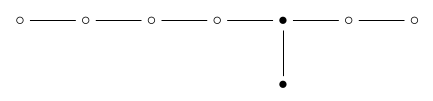and then remove them: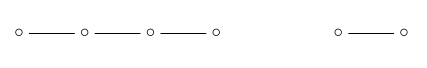leaving me with the Dynkin diagram for $\mathrm{A}_4 \times \mathrm{A}_2$. So, the number of these pairs is

$\begin{array}{ccl} \frac{|W(\mathrm{E}_8)|}{|W(\mathrm{A}_4)| \times |W(\mathrm{A}_2)| \times |W(\mathrm{A}_4)|} &=& \frac{2 \cdot 8 \cdot 12 \cdot 14 \cdot 18 \cdot 20 \cdot 24 \cdot 30}{(2 \cdot 3 \cdot 4 \cdot 5) \times (2 \times 3)}\end{array}$

On the other hand, I already counted the number of 4-simplexes. But just as a reminder: to do this, we remove just the 4-simplex dot from the Dynkin diagram:leaving us with $\mathrm{A}_4 \times \mathrm{A}_1 \times \mathrm{A}_2$:so the number of 4d faces is

$\begin{array}{ccl} \frac{|W(\mathrm{E}_8)|}{|W(\mathrm{A}_4)| \times |W(\mathrm{A}_1)| \times |W(\mathrm{A}_4)|} &=& \frac{2 \cdot 8 \cdot 12 \cdot 14 \cdot 18 \cdot 20 \cdot 24 \cdot 30}{(2 \cdot 3 \cdot 4 \cdot 5) \times 2 \times (2 \cdot 3)} \end{array}$

You can see this is half our previous answer. So, yes: there are two 7-simplexes containing each 4-simplex!

Posted by: John Baez on September 8, 2013 4:13 PM | Permalink | Reply to this

### Re: Integral Octonions (Part 5)

Before I forget:

Puzzle 1. If you double each of the magic numbers and subtract one, then add up the results, you get the dimension of the Lie group corresponding to this Dynkin diagram. Why?

Answer. A self-contained proof can be found in Humphrey’s book Reflection Groups and Coxeter Groups; see Theorem 3.9. Personally I prefer using the fact that the real cohomology of the Lie group $G$ corresponding to the Dynkin diagram is an exterior algebra on generators $x_i$ of degrees $2d_i - 1$. Thus, the highest-degree element in the cohomology of $G$ is the product $x_1 \cdots x_n$, which has degree

$\sum_{i=1}^n (2d_i - 1)$

But the highest degree possible in the cohomology of $G$ is just the dimension of $G$.

Posted by: John Baez on September 5, 2013 11:34 AM | Permalink | Reply to this

### Re: Integral Octonions (Part 5)

It must have been asked before, and I’m sure it’s implicit somehow in the azimuth polytope series, but where does the apparent grading on the dots come from? That is, why should we draw $D_5$ as

            *
/
* - * - *
\
*


    *
\
* - * - *
/
*


???

Posted by: Jesse McKeown on September 5, 2013 7:06 PM | Permalink | Reply to this

### Re: Integral Octonions (Part 5)

That’s a great question! There is no good reason to prefer one grading over another!

The Coxeter complex of a Dynkin diagram (or more precisely Coxeter diagram) treats all dots in an even-handed way. But then, starting from that, you can get different polytopes from choosing different subsets of the dots. I have chosen one particular way to do this in my $\mathrm{E}_8$ example, by taking a subset consisting only of the leftmost dot in the $\mathrm{E}_8$ Dynkin diagram.

Maybe you can see the pattern in this $\mathrm{B}_3$ example. This example is not simply-laced, so I’ll write “3” on an edge when two roots lie at a 120° angle and “4” when they lie at a 135° angle.

Beware: the use of light and dark dots here is different than in the blog article above; now I’m using them to choose which polytope to look at, not to count faces of a fixed polytope.

•—4—o—3—o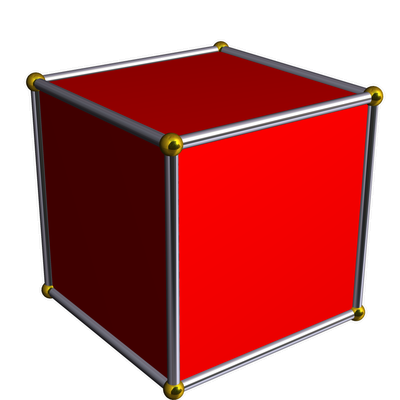cube

•—4—•—3—o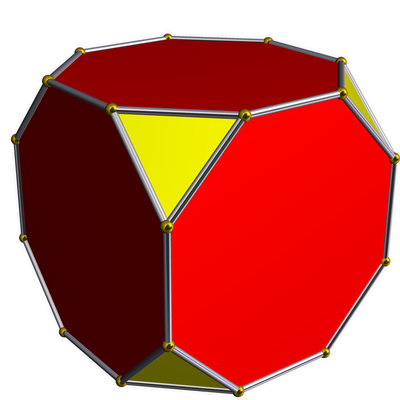truncated cube

o—4—•—3—o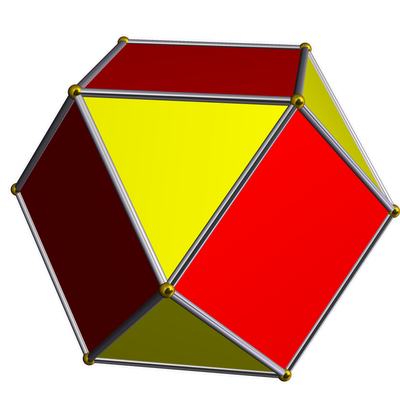cuboctahedron

o—4—o—3—•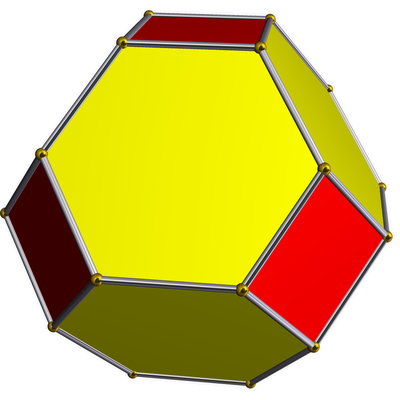truncated octahedron

o—4—o—3—•octahedron

•—4—o—3—•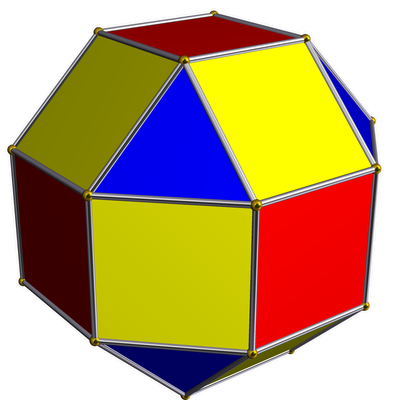rhombicuboctahedron

•—4—•—3—•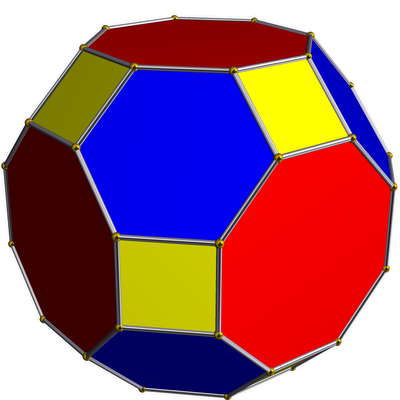truncated cuboctahedron

I’m scared to draw what happens when our subset is the empty set. When we put all the dots in our subset, we get the biggest and fanciest polytope, which is dual to the Coxeter complex:Unfortunately the diagrams above are drawn ‘backwards’ relative to how I drew the $\mathrm{E}_8$ diagram in this post! So, of all the $\mathrm{B}_3$ polytopes shown above, it’s the octahedron:

o—4—o—3—•with the rightmost dot marked, that most resembles the $\mathrm{E}_8$ root polytope, where the leftmost dot of this diagram is marked:If you want to see what happens when we play this game for $\mathrm{E}_8$, go here. Unfortunately there aren’t pictures of all $2^8 - 1 = 255$ polytopes formed by choosing all possible nonempty subsets of the 8 dots—just a few of the most famous ones.

If anyone wants more detail, hop on over to my posts on Azimuth where I’m explaining this stuff in loving detail! There I’m slowly going from 3 dimensions up to 4… while here I’m starting at dimension 8 and aiming for 10.

Here’s the series: part 1, part 2, part 3, part 4, part 5, part 6, part 7, part 8 and part 9. There’s more, but these explain what you’re asking about.

Posted by: John Baez on September 6, 2013 4:41 AM | Permalink | Reply to this

### Re: Integral Octonions (Part 5)

The gosset symmetries go down as far as two dimensions, where E_2 is represented by the body-centred 1:sqrt(7) lattice. The relevant gosset series goes

2, 6, 10, 16, 27, 56, 200 (dec 240),

The product of these numbers gives the order of E_n.

The set of lattices represents (after 3d), the third trigonal group. The lattice A_n is represented by the 60-degree rhombotope, with ‘deep holes’ at x/(n+1) of the long diagonal. The vertex is in a ‘deep hole’ by symmetry.

If you take layers of spheres, of shape A(n-1), and stack them at the first, second or third holes, you get the corresends to the first, second and third trigonal groups (or An, Bn and En respectively). The zeroth one Zn gives a prismatic layer of A(n-1), also counts.

When the trigonal lattices are used to pack spheres of diameter sqrt(2) at the vertices, the volume of the voronii cell is the square root of the number of stations (or standing points), as An = n+1, Bn = 4, En = 9-n. Zn = 2n. The stations are the ‘deep holes’ or centres of cells + the vertices, represents the different places where the next layer is stacked.

The packing efficiency is then 1/volume, is maximum for the first 8 dimensions in a trigonal packing.

In 3, 5, 6 and 7 dimensions, it is possible to have a non-lattice packing as efficient. In 3d, this is the hexagonal close-pack. In 5, 6, and 7 dimensions, it is possible to construct E5, E6 and E7 from layers of Bn (which is the semicubic), by packing these as a lattice. But the layers are far enough apart that one can place the second layer in either position, which allows a non-cubic packing.

In 9 dimensions, the most efficient packing is a quarter-cubic, and if you jiggle it carefully, it will come apart! Strange but true.

Posted by: Wendy Krieger on November 10, 2013 10:09 AM | Permalink | Reply to this

### Re: Integral Octonions (Part 5)

The way to derive the surtope (surface polytope) consist of a polytope is to use the SA rule. The marked nodes are actually edges, and the vertex node is connected to every edge-node.

An S mirror is a ‘surround’ mirror, which reflects a surtope into itself, but changes the bits around.

An A mirror is an ‘around’ mirror, which leaves the surtope unchanged: the surtope is in the mirror.

A W mirror is a ‘wall mirror’, which reflects a surtope onto a different copy: that is the image does not coincide with the original.

The S and A mirrors live inside a room bounded by W mirrors, operate on the same surtope. So if we count the number of rooms, as G/SA, we get the number of surtopes.

The ‘vertex node’ 0, is connected to every marked node. A surtope is made of the vertex-node, and 0 or more mirror nodes, which must be connected to the vertex node.

A polytope like x3o3x5o (the ‘runcinated 3,3,5’), has four nodes, numbered from 1 to 4. The 0 node is connected to 1 and 3.

The vertex is 0. This is directly connected to 1 and 3 (wall nodes), and not connected to 2 and 4. The a symmetry is then of order 4, and the S symmetry is 1, so the count is 14400/4/1 = 3600.

0 = vertex, W=1,3 A=2,4 giving 14400/1/4 = 3600

The edges are 01 and 03. The walls for 01 are 23, and for 03, 234. The A-nodes come to the rest (ie 4, and none), so there are 14400/4 of the former, and 14400/2 of the latter. ie

01 = edge, W=2,3 A=4, giving 14400/2/2 = 3600
03 = edge, W=1,2,4 A=- giving 14400/2/1 = 7200

The hedra (2d patches) are 012, 013, 023, and 024.

012 = triangle, W=3, A=4, giving 14400/6/2 = 1200.
013 = square, W=2,4 A=- giving 14400/4/1 = 3600
023 = triangle, W=1,4 A=- giving 14400/6/1 = 2400
024 = pentagon, W=1,2 A=- giving 14400/10/1 = 1440

The chora (3d patches) are 0123, 0134, and 0234. There are no A-nodes, the unreferenced nodes are all wall-nodes.

0123 = cuboctahedron, giving 14400/24/1 = 600
0134 = pentagonal prism, giving 14400/20/1 = 720
0234 = icosadodecahedra, giving 14400/120/1 = 120.

The tera (4d patches) are 01234.

01234 = cantellated 335, giving 14400/14400/1 = 1.

And there you go. No great mystery.

For something like the twelftycell o3o3o5x, we have 0 is connected to node 4 only, so

surtope consist:

0 = vertex, W = 4, A=1,2,3 14400/1/24 = 600
04 = edge , W = 3, A=1,2 14400/2/6 = 1200
043 = pentagon, W=2, A=1. 14400/10/2 = 720
0432 = dodecahedron, W=1, A=- 14400/120/1 = 120

Terminology follows the polygloss at my web site

http://www.os2fan2.com/

Posted by: Wendy Krieger on November 10, 2013 10:34 AM | Permalink | Reply to this

### Re: Integral Octonions (Part 5)

OK, more notes, mostly for my own reference, on simplex counting. Layra and/or Greg may well have done this already, but I didn’t spot it if so.

1) Vertices.

Roots are either

$\left(\pm1,\pm1,0,0,0,0,0,0\right)$, of which there are $\left(\array{8\\2}\right)\times2\times2=112$

or

$\left(\pm\frac{1}{2},\pm\frac{1}{2},\pm\frac{1}{2},\pm\frac{1}{2},\pm\frac{1}{2},\pm\frac{1}{2},\pm\frac{1}{2},\pm\frac{1}{2}\right)$ with an even number of minus signs (i.e. half the possible choices of sign), of which there are $\frac{1}{2}2^7=128$

$112+128=240$ vertices.

2) Edges

Pick the vertex $\left(1,1,0,0,0,0,0,0\right)$. Adjacent vertices have a dot product of $1$ with this. This means

a) They are all integers, with a $1$ in either first or second place (2 choices) and $\pm1$ (2 choices) in any of the last $6$ places (6 choices). So there are $2\times2\times6=24$ of these.

b) They are all half-integers. There must be $+\frac{1}{2}$ in the first two places, and an even number of minus signs in the other $6$ places. There are $\frac{1}{2}2^6=32$ of these.

This gives $24+32=56$ edges per vertex.

There are $240$ vertices, and $2$ vertices per edge, hence

$\displaystyle\frac{240\times56}{2}=6720$ edges.

3) Triangles

Pick the vertices

$\array{\arrayopts{\collayout{left}} \left(1,1,0,0,0,0,0,0\right)\\ \left(1,0,1,0,0,0,0,0\right) }$

There are $3$ kinds of vertex that have a dot product of $1$ with both of these.

a) All integers with $1$ in the first place and $\pm1$ in any of the last $5$ places. There are $2\times5=10$ of these.

b) All half-integers, with $+\frac{1}{2}$ in the first $3$ places and any even number of minus signs in the last $5$ places. There are $\frac{1}{2}2^5=16$ of these.

c) $\left(0,1,1,0,0,0,0,0\right)$. There is one of these.

So there are $10+16+1=27$ triangles per edge.

There are $6720$ edges, $27$ triangles per edge, and $3$ edges per triangle, hence

$\displaystyle\frac{6720\times27}{3}=60480$ triangles.

4) Tetrahedra

Pick the vertices

$\array{\arrayopts{\collayout{left}} \left(1,1,0,0,0,0,0,0\right)\\ \left(1,0,1,0,0,0,0,0\right)\\ \left(1,0,0,1,0,0,0,0\right) }$

There are $2$ kinds of vertex that have a dot product of $1$ with all of these.

a) All integers with $1$ in the first place and $\pm1$ in any of the last $4$ places. There are $2\times4=8$ of these.

b) All half-integers, with $+\frac{1}{2}$ in the first $4$ places and any even number of minus signs in the last $4$ places. There are $\frac{1}{2}2^4=8$ of these.

So there are $8+8=16$ tetrahedra per triangle.

There are $60480$ triangles, $16$ tetrahedra per triangle, and $4$ triangles per tetrahedron, hence

$\displaystyle\frac{60480\times16}{4}=241920$ tetrahedra.

5) $4$-Simplices

Pick the vertices

$\array{\arrayopts{\collayout{left}} \left(1,1,0,0,0,0,0,0\right)\\ \left(1,0,1,0,0,0,0,0\right)\\ \left(1,0,0,1,0,0,0,0\right)\\ \left(1,0,0,0,1,0,0,0\right) }$

There are $2$ kinds of vertex that have a dot product of $1$ with all of these.

a) All integers with $1$ in the first place and $\pm1$ in any of the last $3$ places. There are $2\times3=6$ of these.

b) All half-integers, with $+\frac{1}{2}$ in the first $5$ places and any even number of minus signs in the last $3$ places. There are $\frac{1}{2}2^3=4$ of these.

So there are $6+4=10$ $4$-simplices per tetrahedron.

There are $241920$ tetrahedra, $10$ $4$-simplices per tetrahedron, and $5$ tetrahedra per $4$-simplex, hence

$\displaystyle\frac{241920\times10}{5}=483840$ $4$-simplices.

6) $5$-Simplices

Pick the vertices

$\array{\arrayopts{\collayout{left}} \left(1,1,0,0,0,0,0,0\right)\\ \left(1,0,1,0,0,0,0,0\right)\\ \left(1,0,0,1,0,0,0,0\right)\\ \left(1,0,0,0,1,0,0,0\right)\\ \left(1,0,0,0,0,1,0,0\right) }$

There are $2$ kinds of vertex that have a dot product of $1$ with all of these.

a) All integers with $1$ in the first place and $\pm1$ in any of the last $2$ places. There are $2\times2=4$ of these.

b) All half-integers, with $+\frac{1}{2}$ in the first $6$ places and any even number of minus signs in the last $2$ places. There are $\frac{1}{2}2^2=2$ of these.

So there are $4+2=6$ $5$-simplices per $4$-simplex.

There are $483840$ $4$-simplices, $6$ $5$-simplices per $4$-simplex, and $6$ $4$-simplices per $5$-simplex, hence

$\displaystyle\frac{483840\times5}{5}=483840$ $5$-simplices.

6) $6$-Simplices

Pick the vertices

$\array{\arrayopts{\collayout{left}} \left(1,1,0,0,0,0,0,0\right)\\ \left(1,0,1,0,0,0,0,0\right)\\ \left(1,0,0,1,0,0,0,0\right)\\ \left(1,0,0,0,1,0,0,0\right)\\ \left(1,0,0,0,0,1,0,0\right)\\ \left(1,0,0,0,0,0,1,0\right) }$

There are $2$ kinds of vertex that have a dot product of $1$ with all of these.

a) All integers with $1$ in the first place and $\pm1$ in any of the last $1$ places (heh!). There are $2\times1=2$ of these.

b) All half-integers, with $+\frac{1}{2}$ in the first $7$ places and any even number of minus signs in the last $1$ places (heh!). There are $\frac{1}{2}2^1=1$ of these.

So there are $2+1=3$ $6$-simplices per $5$-simplex.

There are $483840$ $5$-simplices, $3$ $6$-simplices per $5$-simplex, and $7$ $5$-simplices per $6$-simplex, hence

$\displaystyle\frac{483840\times3}{7}=207360$ $6$-simplices.

7) $7$-simplices

Here is where it gets slightly complicated. Remember that we picked the $5$-simplex

$\array{\arrayopts{\collayout{left}} \left(1,1,0,0,0,0,0,0\right)\\ \left(1,0,1,0,0,0,0,0\right)\\ \left(1,0,0,1,0,0,0,0\right)\\ \left(1,0,0,0,1,0,0,0\right)\\ \left(1,0,0,0,0,1,0,0\right)\\ \left(1,0,0,0,0,0,1,0\right) }$

We found $3$ ways to extend this to a $6$-simplex:

a) $\left(1,0,0,0,0,0,0,1\right)$

b) $\left(1,0,0,0,0,0,0,-1\right)$

c) $\left(\frac{1}{2},\frac{1}{2},\frac{1}{2},\frac{1}{2},\frac{1}{2},\frac{1}{2},\frac{1}{2},\frac{1}{2}\right)$

How can we extend these to a $7$-simplex?

In case a), there is just $1$ way to do it: add point c as well.

In case c), there is just $1$ way to do it: add point a as well.

Obviously these give the same $7$-simplex!

In case b) it can’t be done—we would have to add a half-integer point, but the number of minus signs would have to be odd.

So only $\frac{2}{3}$ of the $6$-simplices extend to a $7$-simplex, and in these cases there is only $1$ $7$-simplex per $6$-simplex. Also, there are $8$ $6$-simplices per $7$-simplex, so overall there are

$\displaystyle\frac{207360\times1}{8}\times\frac{2}{3}=1720$ $7$-simplices.

Note that there are $3$ ways to extend a $5$-simplex to a $6$-simplex, but when extending these $6$-simplices to a $7$-simplex, $2$ of them lead to the same $7$-simplex and $1$ leads to a dead end, so there’s only $1$ way to extend a $5$-simplex to a $7$-simplex.

Posted by: Tim Silverman on March 27, 2015 12:41 PM | Permalink | Reply to this

### Re: Integral Octonions (Part 5)

Of course there’s a typo right at the end: $17280$ $7$-simplices, not $1720$.

Posted by: Tim Silverman on March 27, 2015 12:45 PM | Permalink | Reply to this

### Re: Integral Octonions (Part 5)

OK, a couple more things.

We can see that any $4$-simplex belongs to $2$ $7$-simplices as follows:

Any $4$-simplex can be extended to a $5$-simplex in $6$ different ways, i.e. there is a set of $6$ vertices that can be added.

To get from a $4$-simplex to a $7$-simplex, we have to add $3$ vertices. But once we’ve added $1$ vertex, the next two vertices are forced, because a $5$-simplex lies in a unique $7$-simplex. And obviously adding any one of these vertices to the original $4$-simplex must give a $5$-simplex, so all three vertices belong to the set of $6$. And of course we could have started off by adding another of those $3$ vertices and been forced into the same $7$-simplex.

So the set of $6$ vertices must be partitioned into $2$ sets of $3$, each giving one $7$-simplex.

Second, orthoplexes.

From our $5$-simplex

$\array{\arrayopts{\collayout{left}} \left(1,1,0,0,0,0,0,0\right)\\ \left(1,0,1,0,0,0,0,0\right)\\ \left(1,0,0,1,0,0,0,0\right)\\ \left(1,0,0,0,1,0,0,0\right)\\ \left(1,0,0,0,0,1,0,0\right)\\ \left(1,0,0,0,0,0,1,0\right) }$

we had $3$ ways to get a $6$-simplex:

a) $\left(1,0,0,0,0,0,0,1\right)$ (white)

b) $\left(1,0,0,0,0,0,0,-1\right)$ (black)

c) $\left(\frac{1}{2},\frac{1}{2},\frac{1}{2},\frac{1}{2},\frac{1}{2},\frac{1}{2},\frac{1}{2},\frac{1}{2}\right)$ (white)

Here, a white $6$-simplex lies in a $7$-simplex and an orthoplex, while a black one lies in $2$ orthoplexes.

Now clearly, the $6$-simplex given by a) lies in the orthoplex

$\array{\arrayopts{\collayout{left}} \left(1,\pm1,0,0,0,0,0,0\right)\\ \left(1,0,\pm1,0,0,0,0,0\right)\\ \left(1,0,0,\pm1,0,0,0,0\right)\\ \left(1,0,0,0,\pm1,0,0,0\right)\\ \left(1,0,0,0,0,\pm1,0,0\right)\\ \left(1,0,0,0,0,0,\pm1,0\right)\\ \left(1,0,0,0,0,0,0,\pm1\right) }$

(where we take both signs to give different points of the orthoplex).

But also, so does the $6$-simplex given by b).

And the situation should be symmetric with c) substituted for a).

So we have the following picture:

We have $3$ vertices that we can add to a $5$-simplex to give a $6$ simplex. Let’s call them $w_1$, $w_2$ and $b$.

Adding either $w_1$ or $w_2$ gives a white $6$-simplex, while adding $b$ gives a black $6$-simplex.

There are also $3$ ways to add two of these vertices.

Adding both $w_1$ and $w_2$ gives a $7$-simplex.

Adding $b$ together with $w_1$ forces one orthoplex; adding $b$ together with $w_2$ forces a second orthoplex.

If we start with a $4$-simplex, we have available two different triples of the form $\{w_1,w_2,b\}$

Posted by: Tim Silverman on March 27, 2015 2:32 PM | Permalink | Reply to this

### Re: Integral Octonions (Part 5)

So it’s kind of like a higher-dimensional version of the diagram below (where “7o” is a $7$-orthoplex, “7s” is a $7$-simplex, “6w” is a white $6$-simplex, “6b” is a black $6$-simplex, and “5” is a $5$-simplex).

Layer 1 7s 7o 7o 6w 6w 6b 5

Posted by: Tim Silverman on March 27, 2015 2:53 PM | Permalink | Reply to this

### Re: Integral Octonions (Part 5)

OK, now I’m curious about the dual of the $E_8$ polytope.

Dual to the $7$-simplices and $7$-orthoplexes, we have two types of point, $s$-points and $o$-points. Dual to the black and white $6$-simplices we have two types of line, $b$-lines each joining two $o$-points, and $w$-lines each joining an $o$-point to an $s$-point.

The $5$-simplices then correspond to isoceles triangles, with two $o$-points and an $s$-point, one $b$-line and one $w$-line.

What about the $4$-simplices? Their duals are polyhedra, whose faces are isoceles triangles.

OK, we know that each $4$-simplex lies in $6$ $5$-simplices, so this polyhedron has $6$ faces. And the faces are triangles, meeting along their edges, so there are $\displaystyle\frac{6\times3}{2}=9$ edges.

But $F-E+V=2$, so there must be $5$ vertices.

The figure that I think fits is the trigonal bipyramid: an equilateral triangle of $o$-points joined by $b$-edges, with one $s$-point above and one below the plane of the triangle, with $3$ $w$-edges from each $s$-point, joining it to the $3$ $o$-points. That gives $3$ $b$-edges and $6$ $w$ edges.

So each $4$-simplex should lie in $3$ black $6$-simplices and $6$ white ones?

Posted by: Tim Silverman on March 27, 2015 3:38 PM | Permalink | Reply to this

### Re: Integral Octonions (Part 5)

Thanks for joining the fun, Tim! I’m sorry that you weren’t in on it back when I was actively thinking about this stuff. But I will return someday.

Posted by: John Baez on March 27, 2015 4:32 PM | Permalink | Reply to this

### Re: Integral Octonions (Part 5)

Oh, well, the timing is so often wrong, and I’m busy when the topic comes up the first time. But since I started thinking about this again, and there was already a dormant thread, I thought I might as well put my thoughts out in the open in case anyone found it useful at some point.

Posted by: Tim Silverman on March 27, 2015 9:01 PM | Permalink | Reply to this

### Re: Integral Octonions (Part 5)

OK, the symmetry seems right too. If we take the $E_8$ dynkin diagram and delete everything from the $4$-simplices downward, we get left with

a) a copy of the $A_1$ diagram containing the $7$-simplices, representing the reflection across the equatorial plane which swaps the two $s$ points, and

b) a copy of the $A_2$ diagram with the $7$ orthoplexes and the black $6$-simplices, representing the triangular symmetry of the equatorial plane (determined by a flag consisting of an equatorial (ie $o$-) point and an equatorial (ie $b$-) line).

Good.

What about the duals of the tetrahedra? These are $4$-polytopes, all identical. Let’s try to characterise them.

Since there are $10$ $4$-simplices containing a given tetrahedron, there are $10$ trigonal-bipyramidal cells in this polytope.

Since each has $6$ faces, and each face is shared by $2$ cells, there are $\displaystyle\frac{10\times6}{2}=30$ triangular faces.

What about edges? There are two types, and I’m not sure how many cells there are around each type. Probably $3$, but let’s check.

In $4$ dimensions, $C-F+E-V=0$, so $V=C-F+E=10-30+E=E-20$.

Since we already have $5$ vertices in a single cell, and there must be at least one more, we must have $E\ge26$.

There are $6$ $w$-edges and $3$ $b$-edges per cell, and $10$ cells, so the total number of edges must be of the form $\displaystyle\frac{10\times6}{a}+\displaystyle\frac{10\times3}{a}$, with $a$ and $b$ being factors fo $60$ and $30$ respectively, and equal to at least $3$ (since we must have more than $2$ cells around an edge.

To fulfill these requirements, it turns out that $a=20$ and $b=3$ or $b=5$. But there isn’t room to fit $5$ cells around an equatorial edge, so $b=3$ and

$E=\displaystyle\frac{90}{3}=30$, including $20$ of $w$ type and $10$ of $b$ type.

Hence $V=10$. That means $4$ $s$-vertices and $6$ $o$-vertices.

Since there are $10$ cells and $10$ vertices, and $5$ vertices per cell, there must be $5$ cells around each vertex, at least on average.

If we take the $E_8$ dynkin diagram and remove everything from $3$-simplices down, we get the $A_4$ diagram. So the symmetry group of the $4$-polytope dual to a tetrahedron is the symmetric group $S_5$. Which is … surprising. It’s the same as that of the simplex in $4$ dimensions, too. And there should be a duality swapping the two types of points ($s$ and $o$), and also swapping the equatorial edges with the cells … which is a bit mind-boggling … at least to me.

Posted by: Tim Silverman on March 27, 2015 5:14 PM | Permalink | Reply to this

### Re: Integral Octonions (Part 5)

Another fun thing I think I forgot to mention earlier is that you can tile 8-dimensional space with the $\mathrm{E}_8$ root polytope together with the 8-simplex! On Wikipedia, the $\mathrm{E}_8$ root polytope is called $2_{41}$, and the uniform tesselation you can build with this polytope and the 8-simplex is called the $2_{51}$ honeycomb. There’s a lot of information there.

Posted by: John Baez on March 27, 2015 6:12 PM | Permalink | Reply to this

### Re: Integral Octonions (Part 5)

OK, I realised I was feeling a bit vague about the dimensions of the trigonal bipyramid. So I should calculate the lengths of the two kinds of edges.

1) $w$-edges between an $s$-point and an $o$-point. We need suitable examples of the latter.

But we’ve got these from earlier. The white $6$-simplex given by

$\array{\arrayopts{\collayout{left}} \left(1,1,0,0,0,0,0,0\right)\\ \left(1,0,1,0,0,0,0,0\right)\\ \left(1,0,0,1,0,0,0,0\right)\\ \left(1,0,0,0,1,0,0,0\right)\\ \left(1,0,0,0,0,1,0,0\right)\\ \left(1,0,0,0,0,0,1,0\right)\\ \left(1,0,0,0,0,0,0,1\right) }$

can be extended to a $7$-simplex by adding

$\left(\frac{1}{2},\frac{1}{2},\frac{1}{2},\frac{1}{2},\frac{1}{2},\frac{1}{2},\frac{1}{2},\frac{1}{2}\right)$

and also lives in the $7$-orthoplex given by

$\array{\arrayopts{\collayout{left}} \left(1,\pm1,0,0,0,0,0,0\right)\\ \left(1,0,\pm1,0,0,0,0,0\right)\\ \left(1,0,0,\pm1,0,0,0,0\right)\\ \left(1,0,0,0,\pm1,0,0,0\right)\\ \left(1,0,0,0,0,\pm1,0,0\right)\\ \left(1,0,0,0,0,0,\pm1,0\right)\\ \left(1,0,0,0,0,0,0,\pm1\right) }$

Now the centroid of the first is

$\frac{1}{8}\left(7\frac{1}{2},1\frac{1}{2},1\frac{1}{2},1\frac{1}{2},1\frac{1}{2},1\frac{1}{2},1\frac{1}{2},1\frac{1}{2}\right)$

i.e.

$\left(\frac{15}{16},\frac{3}{16},\frac{3}{16},\frac{3}{16},\frac{3}{16},\frac{3}{16},\frac{3}{16},\frac{3}{16}\right)$

And the centroid of the first is obviously

$\left(1,0,0,0,0,0,0,0\right)$

Subtracting the second from the first gives

$\left(\frac{-1}{16},\frac{3}{16},\frac{3}{16},\frac{3}{16},\frac{3}{16},\frac{3}{16},\frac{3}{16},\frac{3}{16}\right)$

of length

$\frac{1}{16}\sqrt{1+7\times3^2}=\frac{8}{16}=\frac{1}{2}$

So $w$-lines are of length $\frac{1}{2}$.

Now, since orthoplex centres lie on a line with lattice points of length $2$, we can get the angle between two neighbours by maximising the cosine of the angle between them, i.e. maximising their dot product.

Picking $\left(1,1,1,1,0,0,0,0\right)$, no half-integer point has a dot product with this of more than $2$, but, e.g. $\left(0,1,1,1,1,0,0,0\right)$ has a dot product with it of $3$. Since the lengths of the vectors are $2$, the angle between them is $cos^{-1}\frac{3}{4}$ (as Greg says in several places).

From our example above, $\left(1,0,0,0,0,0,0,0\right)$, we see that orthoplex centres are at distance $1$ from the origin, so are separated by the third edge of a triangle with two edges of length $1$, with an angle of $cos^{-1}\frac{3}{4}$ between them.

So the distance is $2sin\left(\frac{1}{2}cos^{-1}\frac{3}{4}\right)=2\sqrt{\frac{1}{2}\left(1-cos\left(cos^{-1}\frac{3}{4}\right)\right)}=\displaystyle\frac{\sqrt{2}}{2}$

So in a triangle $s$-$o$-$o$, the sides $s$-$o$ are of length $\frac{1}{2}$ and the sides $o$-$o$ are of length $\displaystyle\frac{\sqrt{2}}{2}$. This means that the angles $\angle s o o$ are $45^\circ$ while the angle $\angle o s o$ is $90^\circ$.

So the $s$-vertices of the trigonal bipyramid are like the vertices of cubes. That is, take a cube of side $\displaystyle\frac{\sqrt{2}}{2}$. Pick a vertex. The three vertices adjacent to it form a triangle lying in a plane. Slice off the top of the cube along that plane. Take a reflection of this cubic cap through its base and glue it to the original (along their common base). That’s the solid dual to the $4$-simplex.

Posted by: Tim Silverman on March 27, 2015 9:29 PM | Permalink | Reply to this

### Re: Integral Octonions (Part 5)

Tush! Obviously every $o$-vertex is incident with every other $o$-vertex.

Posted by: Tim Silverman on March 27, 2015 11:11 PM | Permalink | Reply to this

### Re: Integral Octonions (Part 5)

OK, back to the $4$-polytope. Let’s think about symmetry.

The fact that the diagram for this polytope apparently has a duality between $s$-points and $o$-points suggests that they ought to be equal in number, i.e. $5$ of each. That would mean different numbers of cells around the two types.

We have $2$ $s$-points per cell, and $10$ cells, but only $5$ $s$-points in total, then we would have $\displaystyle\frac{10\times2}{5}=4$ cells around each $s$-point.

We have $3$ $o$-points per cell, and $10$ cells, but only $5$ $o$-points in total, so we would have $\displaystyle\frac{10\times3}{5}=6$ cells around each $o$-point.

OK, I don’t know if this is right, but let’s run with it. Lets pick one cell, call it the “central” cell, and look at the cells around its vertices.

If we have $4$ cells around an $s$-vertex, then they are just the central cell together with the cells attached to its $3$ faces surrounding the vertex. Since the vertex figure of an $s$-point in the $3$-dimensional cell is a triangle, and all the cells and edges are the same there, I guess the vertex figure in the $4$-dimensional polytope is a regular tetrahedron. And so there are $4$ edges emanating from it, $3$ of them incident with the central cell, and one not.

If we have $6$ cells around an $o$-vertex, then they are the central cell, the $4$ cells attached to the $4$ adjacent faces, plus one more. Since the $o$-vertex is incident with $4$ edges of the central cell, its vertex figure in $3$ dimensions is a square (or at least a sort of rhombus—$2$ edges go to $s$-vertices and $2$ to $o$-vertices). So I guess the vertex figure in the $4$-polytope is a cube, or at least a rhombic parallelepiped. So an $o$-vertex is incident with $8$ edges of the $4$-polytope (corresponding to the $8$ vertices of the cube), $4$ of them lying in the central cell and $4$ not.

Let’s count cells incident with vertices of the central cell.

We have the central cell itself. We have $6$ cells sharing faces with it. At each of the $3$ equatorial ($o$) points we have another cell sharing the vertex but no faces. This totals $1+6+3=9$ cells, so there is just one cell which shares no vertices with the central cell.

So the $10$ cells fall into $5$ pairs, which goes some why to explaining why they might behave like the $5$ cells of a $4$-simplex.

OK, now each $s$-vertex is incident with $4$ edges ($3$ of them in the central cell, the other one not), and these edges are all $w$-edges and are incident also with one $o$-vertex each. So each $s$-vertex is connected to $4$ $o$-vertices. If there really are $5$ $o$-vertices in total, then each $s$-vertex is non-incident with just $1$ $o$-vertex, giving a pairing between vertices which goes some way to explaining how the $10$ vertices might behave like the $5$ cells of a $4$ simplex.

(We can see the same thing by picking an $o$-vertex. It is connected to $8$ other vertices, half of them $o$-vertices and half, i.e. $4$ of them $s$-vertices. So it is non-incident with $1$ $s$ vertex. Also with $1$ $o$-vertex.)

OK, that’ll do for now.

Posted by: Tim Silverman on March 27, 2015 11:05 PM | Permalink | Reply to this

### Re: Integral Octonions (Part 5)

Every $o$-vertex is incident with every other $o$-vertex, and I should go to bed now.

Posted by: Tim Silverman on March 27, 2015 11:13 PM | Permalink | Reply to this

### Re: Integral Octonions (Part 5)

OK, well, it turns out that I can’t count, and there is no pairing on the $10$ cells. However, I have now worked out the combinatorics of it all.

There are $5$ $s$-vertices, $\{a,b,c,d,e\}$. There are $2$ $s$-vertices in each cell. Let’s say that two $s$-vertices are “connected” by the cell they share. But we have seen that every $s$-vertex lies in $4$ cells, so every $s$-vertex is connected to every other.

There are $5$ $o$-vertices, $\{A,B,C,D,E\}$. There are $2$ $o$-vertices for every black edge. Let’s say that two $o$-vertices are “connected” by the black edge they are in. But we have seen that every $o$-vertex lies in $8$ edges, $4$ of them black and $4$ white, so every $o$-vertex is connected to every other.

Finally, let us say that an $s$-vertex and an $o$-vertex are “connected” precisely when they do not share any white edges. We saw above that each $s$-vertex is connected to precisely one $o$-vertex. So let’s indicate this connection by change of capitalisation: $a$ is connected to $A$, etc.

Now we have that every cell is just a pair of $s$-vertices, e.g. $\left(ab\right)$. That is why there are $\left(\array{5\\2}\right)=10$ cells.

And every black edge is just a pair of $o$-vertices, e.g. $\left(AB\right)$. That is why there are $\left(\array{5\\2}\right)=10$ black edges.

A white edge connects an $s$-vertex and an $o$-vertex, and any two can be connected unless they have the same letter. So each of the $5$ $s$-vertices can be paried to $4$ of the $o$-vertices, which is why there are $5\times4=20$ white edges.

A face contains an $s$-vertex and $2$ $o$-vertices, neither of which can share its letter (since they are connected to it by white edges). So there are $5\times\left(\array{4\\2}\right)=30$ faces.

A face lies in $2$ cells. E.g. $a B C$ lies in $a d$ and $a e$. The second $s$-vertex of the cell obviously can’t coincide with $a$ (which we’ve already counted) or with $b$ or $c$ (because $B$ and $C$ lie in the face and therefore in the cell, and hence must be connected to the other $s$-vertex by a white edge, and hence can’t share its letter).

An $o$-vertex lies in $6$ cells. It is connected by a white line to every $s$-vertex but one, and hence lies in all the cells determined by the $\left(\array{4\\2}\right)=6$ pairs draw from the set of $4$.

It is patently obvious that this system has $S_5$ symmetry. There is also obviously a duality which swaps $s$-points with $o$-points, and hence also swaps pairs of $s$-points (i.e. cells) with pairs of $o$-points (i.e. black edges). That accounts for the duality of the $A_4$ dynkin diagram.

Posted by: Tim Silverman on March 28, 2015 8:46 AM | Permalink | Reply to this

### Re: Integral Octonions (Part 5)

OK, now let’s look at the actual geometry.

The $5$ $o$-vertices are each connected to each other by black edges, and all the black edges are identical. The only way this is possible in $4$-dimensional space is if they lie at the vertices of a $4$-simplex.

Likewise, the $5$ $s$-vertices are each connected to each other by a cell, and all the cells are identical. So they also lie at the vertices of a $4$-simplex.

And these $4$-simplices are surely dual to each other—apart from an overall scaling. The reasons are:

a) Since they share the same $S_5$ symmetry, the two simplices must be either rescaled versions of each other, or rescaled versions of each others’ duals.

b) If they are dual, then $4$ of the $o$-vertices lies exposed to each $s$-vertex for connection by a white edge, while the $5$th $o$-vertex lies on the other side of the simplex and is hidden from view of the $s$-vertex. The alternative would require the white edges to cross through the interior.

c) The duality of the dynkin diagram, which corresponds to Poincaré duality of the $4$-simplex, swaps they two kinds of point.

So the remaining thing we need to understand is the relative size of the two $2$-simplices, and here there is a crucial restraining factor: the $3$-dimensionality of the bipyramids. We can see this by looking at the $2$- and $3$-dimensional analogues of the pair of $4$-simplices.

Here is the $2$-dimensional analogue:

The blue dots represent $s$-vertices and the red dots represent $o$-vertices. The edges of the outer triangle represent white edges, and the edges of the inner triangle represent black edges.

Here is the analogue of the bipyramid:

It isn’t exactly a bipyramid—it’s a bi-line segment!

Crucially, the two halves fit into a $1$-dimensional line, and this forces the blue triangle to be exactly twice the size of the red triangle, otherwise this figure would have a kink in it and would need $2$ dimensions to fit it in.

Here is the $2$-dimensional analogue:

Layer 1

Again, the blue dots represent $s$-vertices and the red dots $o$-vertices. This time the two simplices are the same size, and the overall polyhedron is a cube.

Each face is a $2$-dimensional analogue of the bipyramid: it is a bi-triangle.

Layer 1

In general, this sort of figure is a rhombus, but in this particular case it is a square.

Now here is the trigonal bipyramid.

Layer 1

In the bi-line, each blue dot is connected to $1$ red dot, and the line between the blue dots passes through the red dot.

In the bi-triangle, each blue dot is connected to $2$ red dots, and the line between the blue dots passes through the midpoint of the edge containing the red dots.

In the bi-pyramid, each blue dot is connected to $3$ red dots, and the line between the blue dots passes through the midpoint of the triangle containing the red dots.

This forces the blue $4$-simplex to be smaller than the red simplex (by a factor of $\sqrt{\frac{2}{3}}$).

Posted by: Tim Silverman on March 29, 2015 2:38 PM | Permalink | Reply to this

### Re: Integral Octonions (Part 5)

I wasn’t going to carry on into $5$ dimensions, and work out the dual of the triangles, but it turns out to be simpler than I thought. Cutting off the leftmost $3$ points of the dynkin diagram for $E_8$ leaves us with the diagram for $D_5$. And indeed Wikipedia’s article on the $2_{41}$ polytope tells us that the figure for $o$-points only is a $5$-orthoplex. Let’s work this out.

The $5$-orthoplex has $10$ points, which we can coordinatise as:

$\left(\pm1,0,0,0,0\right)$ and permutations.

We want to find $4$-simplices in here. We can’t have two vectors with $\pm1$ in the same position, so the $5$ points of the $4$-simplex need to have, between them, a $1$ in every place. The only difference is the signs. Every combination of signs gives a $4$-simplex, so there are $2^5=32$ $4$-simplices in a $5$-orthoplex.

However, we saw earlier that there are only $16$ tetrahedra per triangle, hence only $16$ of our $4$-simplices in a $5$-orthoplex of $o$-points. So they will be in alternating positions—e.g. we only choose $4$-simplices with an even number of minus signs. And this makes sense—the symmetry group is $D_5$, which is not all the symmetries of the orthoplex (that would be $B_5$) but only half of them.

We now have to place the $s$-points. We know where they are because the lie in rescaled duals of the $4$-simplices. I won’t do the calculation here (it’s not very exciting), but it turns out that unlike the $o$-points, where there is a lot of sharing between $4$-simplices (at the rate of one $o$-point shared by $8$ of them), there is no sharing of $s$ points. Each of the $5$ points of each of the $16$ $4$-simplices is different, and they lie on the $80$ midpoints of the edges of a $5$-cube, which is dual (after rescaling) to the $5$-orthoplex.

So the $s$-points have gone from being half as numerous as $o$-points in the triangles, to $\frac{2}{3}$ as numerous in the bipyramids, to equally numerous in the $4$-simplices, to now $8$ times as numerous, an advantage they will maintain to the end.

At this point things get more complicated. Because we have passed a branch point, there are now $2$ types of $5$-dimensional figures of $o$-points—the orthoplexes we have described, and also $5$-simplices. In $6$ dimensions there are $E_6$-polytopes and $6$-simplices, and in $7$ dimensions there are $E_7$-polytopes and $7$-simplices (just for $o$-points alone). So I think I really will leave it there.

Posted by: Tim Silverman on March 31, 2015 8:37 AM | Permalink | Reply to this

### Re: Integral Octonions (Part 5)

You make things unnecessarily hard. It is quite easy to construct the consist of any polytope on the Coxeter diagram, using a rule I found back in the seventies.

The vertex is another node, connected to every marked node.

1—2—3—4—5—6—7 8 ———-/

Node $is connected to 1. Node 8 is connected to 5. These are mirrors. The ‘s’ mirrors are directly connected to the$ node, and serve to place a reflected copy of the surtope on itself.

The ‘a’ mirrors are not connected to the $node, and reflect the surtope onto itself without altering it. They happen around the surtope. The ‘w’ mirrors reflect the surtope to a different copy of the surtope. Since s and a reflect a surtope onto itself, the cumulative order of these is the symmetry of the ‘room’ containing the surtope, while the ‘w’ (wall) surtopes moves through a wall to a different surtope. This is done in base 120, I don’t have the decimal translation on me. S - W - A S*A G/SA G=3.4372.0000 vertex$ - 1 - 2345678 1*181.7200 200

edge

$1 - 2 - 345678 2*3.7200 5600 2d (hedra)$12 - 3 - 45678 6 * 1600 8.4800

3d (chora)

$123 - 4 - 5678 24*100 16.9600 4d (tera)$1234 - 5 - 67 8 100*12 33.7200

5d (peta)

$12345 - 68 - 7 600*2 33.7200 6d (ecta)$123456 - 78 - x 42001 9.7200 $123458 - 6 - 7 42002 4.9600 7d (zetta)$1234567 - 8 - a 2.96001 1.2400 $1234568 - 7 - b 22.48001 1800 8d (yotta)$12345678 - x - x 3.4324.0000 1

You can see we do two different ecta, because there are two different connected animals containing seven nodes. They are the same, but they are simplex/cross margins, and cross/cross margins (lying in a mirror ‘7’).

The 7d zetta lie perpendicular to a node. In practice, all nodes produce faces, but these are generally zero-height prisms, and hence disappear. Here we have a=simplex, b=cross polytope.

Posted by: Wendy Krieger on January 24, 2016 8:57 AM | Permalink | Reply to this

Post a New Comment